# Clumpy hotspots

Read an article by Tim Hart the other day (part of a special issue I will have an article in as well here soon). In it he evaluated hot spot methods not only by how well they forecast crime, but also by the clumpiness of the hot spot method. Some hot spot methods, such as risk terrain modeling (Caplan et al., 2020; Fox et al., 2021), machine learning models (Wheeler & Steenbeek, 2020), or self-exciting point process models (Mohler et al., 2018) can by their nature produce discontinuous hot spots. Here is an example of a RTM map I made in Yoo & Wheeler (2019) for homeless related crime in Los Angeles, and you can see this is quite spotty in the ups/downs in the high risk areas:Other hot spot methods, like hierarchical clustering (Wheeler & Reuter, 2020) or kernel density maps however this is not as big an issue. Here is an example kernel density map also from Yoo & Wheeler (2019) based on the same data:So you can see how the hot spots in the kernel density map are spatially contiguous, whereas the RTM example can be little hot spots all over the jurisdiction. So it is obviously easier to patrol a single contiguous area than many islands over the entire jurisdiction. So it may make sense to trade off a contiguous area that captures somewhat fewer crimes than speckled areas that are all over the map.

Adepeju et al. (2016) was the first to use a particular statistic, the clumpiness index, to evaluate different hot spot methods. Their figure below is a pretty good depiction of the idea – count up the number of internal edges to a hot spot (when a hot spot grid-cell neighbors another hot spot), and the number of external edges. Then it is just a particular formula to make the index range from -1 to 1 given different sized hot spots.So here I flip this idea on its head abit – instead of using a particular hot spot technique and see its clumpiness, I formulate a linear program given a prediction to trade off a smaller number of predicted crimes in the hot spot vs making the hot spot areas more clumpy. I illustrate my clumpy hot spots using just prior data to predict future data, in particular thefts from motor vehicles in Raleigh North Carolina.

I have posted the data/code on github here. It is a bit too long to embed the code directly in the blog post, but just see the `00_PrepData.py` file. The crime data and Raleigh border I downloaded from the Raleigh open data website.

# A Linear Program to Clump Hot Spots

So for some quick and dirty math in text, the linear program I formulate is:

``````Maximize { Sum[ theta*S_i*Crime_i + (1 - theta)*E_i ] }
Subject To:
1) Sum( S_i ) = k
2) E_i <= Sum(S_n for n in neighbors(i) ) for each i
3) E_i <= S_i for each i
4) S_i element of {0,1}, E_i >= 0 (and can be continuous)``````

The idea behind this is that if `theta=1`, this is the same as just taking whatever your input areas are and ranking them to pick the top `k` areas. So if you have 10000 500 by 500 foot grid cells as your spatial units of analysis, and you wanted the top 1% of the city, that is 100 grid cells. So you would choose `k=100` in that scenario. `Crime_i` here I use as prior counts of crime in the grid cell, but it could be the predicted value from whatever model as well. That is the first constraint in this model approach – you need to choose the total area you want. `S_i` are the decision variables for the final selected hot spot areas.

The second and third constraints determine the values for the second set of decision variables, `E_i`. These are the decision variables that encode the interconnected links when a selected grid cell touches another grid cell. Constraint 2 sets `E_i` to the total number of neighbors of `i` that are selected, except constraint 3 says if `S_i` is 0 `E_i` needs to be 0 as well.

In this formulation, `S_i` need to be integer variables, but the `E_i` are defined by the sum of `S_i`, so they can be continuous. In this formulation if you have N grid cells (or whatever spatial units of analysis), this results in `2*N` decision variables, and `2*N + 1` constraints. You could maybe save a few constraints here by working with an undirected graph instead of a directed one (in essence this double counts, a-b and b-a would count as two links). But this will just make it `1.5*N` constraints instead of `2*N`. So not a big deal probably. I did have some issues solving this using pulps default coin/GLPK solver. But CPLEX solved it no problem. (My example is a total of 20,986 500 by 500 foot grid cells, and I use rook contiguity like the Adepeju article as well. And using CPLEX it solves the models in just a few seconds.)

In this formulation you can think of `theta` as trading off crimes in the hot spot vs interior edges. So imagine you had `theta=0.9`, and you had a solution with 200 crimes and 100 interior edges. The objective function in that scenario would be `0.9*200 + 0.1*100 = 190`. Now imagine you had an alternative scenario with 190 crimes, but 200 internal edges, the objective function would be `0.9*190 + 0.1*200 = 191`. So you are saying, it is ok to have hot spots capture a smaller number of crimes, if they are more connected.

# Normal Hotspots vs Clumpy Ones in Raleigh

The open data I use for Raleigh, North Carolina for the NIBRS dataset goes back to June 2014, and has data updated in the beginning of March 2021. I pull out larcenies from motor vehicles, and for the historical train dataset use car larcenies from 2014 through 2019 (n = 17,681). For the test dataset I use car larcenies in 2020 and what is available so far in 2021 (n = 3,376). Again these are grid cells generated over the city boundaries at 500 by 500 foot intervals. For illustration I grab out the top 1% of the city (209 grid cells). I use a train/test dataset as out of sample test data will typically result in reduced predictions. Here are the PAI stats for train vs test when selecting the top 1%.For all subsequent selections I always use the historical training data to select the hot spots, and the test dataset to evaluate the PAI.

If we do the typical approach of just taking the highest crime grid cells based on the historical data, here are the results both for the PAI and the CI (clumpy index). For those not familiar, PAI is `% Crime Capture/% Area`, so if the denominator is 1%, and the PAI (for the test data) is 17, that means the hot spots capture 17% of the total thefts from vehicles. The CI ranges from -1 (spread apart) to 1 (entirely clustered). Here it is just over 0, suggesting these are basically randomly distributed in terms of clustering.You may think that almost spatial randomness in terms of clumping seems at odds with that crime clusters! But it is not really – a consistent relationship with crime hot spots is that they are intensely localized, and often you can go down the street and be in a low crime area (Harries, 2006). The same idea when people say high crime neighborhoods often are spotty interior – they tend to have mostly low crime areas and just a few specific hot spots.

OK, so now to show off my linear program. So what happens if we use `theta=0.9`?The total crime numbers are here for the historical data, and it ends up capturing the exact same number of crimes as the select top 1% does (3,664). But, it switches the selection of one of the areas. So what happens here is that we have ties – even with basically little weight assigned to the interior connections, it will prioritize tied crime areas to be connected to other chosen hot spots (whereas before the ties are just random in the way I chose the top 1%). So if you have many ties at the threshold for your hot spot, this is a great way to prioritize particular tied areas.

What happens if we turn down theta to 0.5? So this is saying you would trade off one for one – one interior edge is equal to one crime.You can see that it changed the selections slightly more here, traded off 24 areas compared to the original just rank solution. Lets check out the map and the CI:The CI value is now 0.17 (up from 0.08). You can see some larger blobs, but it is still pretty spread apart. But the reduction in the total number of crimes captured is pretty small, going from a PAI of 17 to now a PAI of 16. How about if we crank down theta even more to 0.2?This trades off a much larger number of areas and total amount of crime – over half of the chosen grid cells are flipped in this scenario. In the subsequent map you can see the hot spots are much more clumpy now, and have a CI of 0.64.The PAI of 12.6 is a bit of a hit as well, but is not too shabby still. I typically take a PAI of 10 to be the ballpark of what is reasonable based on Weisburd’s Law of Crime Concentration – 5% of the areas contain 50% of the crime (which is a PAI of 10).

So this shows one linear programming approach to trade off clumpy chosen areas vs disconnected speckles over the map. It may be the case though that other approaches are more reasonable, such as using some type of clustering to begin with. E.g. I could use DBSCAN on the gridded predicted values (Wheeler & Reuter, 2020) as see how clumpy those hot spots are. This approach is nice though if you have a fixed area you want to cover though.

# Why Raleigh?

For a bit of personal news, I will be moving to the Raleigh area here shortly. I recently negotiated to be 100% remote at my job – so I will still be at HMS (or since we were recently purchased I might be employed by Gainwell I guess by the time I move). So looking forward to the new adventure back on the east coast but still in more temperate climates than PA or NY!

# Transforming predicted variables in regression

The other day on LinkedIn I made a point about how I think scikits `TransformedTargetRegressor` is very likely to mislead folks. In fact, the example use case in the docs for this function is a common mistake, fitting a model for `log(y)`, then getting predictions `phat`, and then simply exponentiating those predictions `exp(phat)`.

On LinkedIn I gave an example of how this is problematic for random forests, but here is a similar example for linear regression. For simplicity pretend we only have 3 potential residuals (all equally likely), either a residual of -1, 0, or 1.

Now pretend our logged prediction is 5, so if we simply do `exp(5)` we get about `148`. Now what are our predictions is we consider those 3 potential residuals?

``````Resid  Pred-Resid Modified_Pred LinPred
-1     5 - -1        exp(6)     403
0     5 -  0        exp(5)     148
1     5 -  1        exp(4)      55``````

So if we take the mean of our `LinPred` column, we then get a prediction of about `202`. The prediction using this approach is much higher than the naive approach of simply exponentiating 5. The difference is that the exp(5) estimate is the median, and the above estimate taking into account residuals is the mean estimate.

While there are some cases you may want the median estimate, in that case it probably makes more sense to use a quantile estimator of the median from the get go, as opposed to doing the linear regression on `log(y)`. I think for many (probably most) use cases in which you are predicting dollar values, this underestimate can be very problematic. If you are using these estimates for revenue, you will be way under for example. If you are using these estimates for expenses, holy moly you will probably get fired.

This problem will happen for any non-linear transformation. So while some transformations are ok, in scikit for example minmax or standardnormal scalars are ok, things like logs, square roots, or box-cox transformations are not. (To know if it is a linear transformation, if you do a scatterplot of original vs transformed, if it is a straight line it is ok, if it is a curved line it is not!)

I had a friend go back and forth with me for a bit after I posted this. I want to be clear this is not me saying the model of `log(y)` is the wrong model, it is just to get the estimates for the mean predictions, you need to take a few steps. In particular, one approach to get the mean estimates is to use Duan’s Smearing estimator. I will show how to do that in python below using simulated data.

# Example Duan’s Smearing in python

So first, we import the libraries we will be using. And since this is simulated data, will be setting the seed as well.

``````######################################################
import pandas as pd
import numpy as np
np.random.seed(10)

from sklearn.linear_model import LinearRegression
from sklearn.compose import TransformedTargetRegressor
######################################################``````

Next I will create a simple linear model on the log scale. So the regression of the logged values is the correct one.

``````######################################################
# Make a fake dataset, say these are housing prices
n = (10000,1)
error = np.random.normal(0,1,n)
x1 = np.random.normal(10,3,n)
x2 = np.random.normal(5,1,n)
log_y = 10 + 0.2*x1 + 0.6*x2 + error
y = np.exp(log_y)

dat = pd.DataFrame(np.concatenate([y,x1,x2,log_y,error], axis=1),
columns=['y','x1','x2','log_y','error'])
x_vars = ['x1','x2']

# Lets look at a histogram of y vs log y
dat['y'].hist(bins=100)
dat['log_y'].hist(bins=100)
######################################################``````

Here is the histogram of the original values: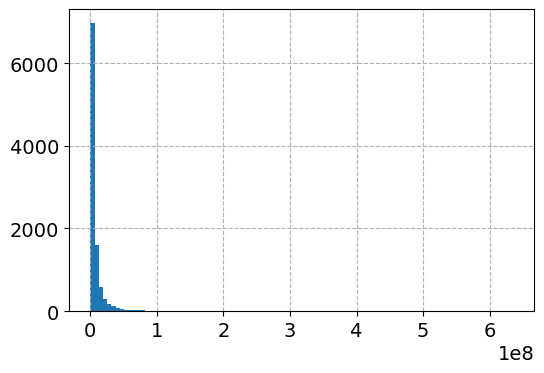And here is the histogram of the logged values: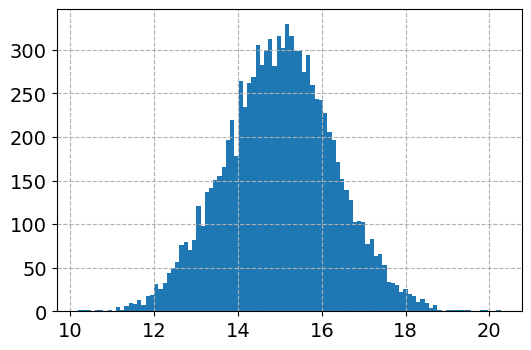So although the regression is the conditional relationship, if you see histograms like this I would also by default use a regression to predict `log(y)`.

Now here I do the same thing as in the original function docs, I fit a linear regression using the log as the function and exponential as the inverse function.

``````######################################################
# Now lets see what happens with the usual approach
tt = TransformedTargetRegressor(regressor=LinearRegression(),
func=np.log, inverse_func=np.exp)
tt.fit(dat[x_vars], dat['y'])
print( (tt.regressor_.intercept_, tt.regressor_.coef_) ) #Estimates the correct values

dat['WrongTrans'] = tt.predict(dat[x_vars])

dat[['y','WrongTrans']].describe()
######################################################``````

So here we estimate the correct simulated values for the regression equation: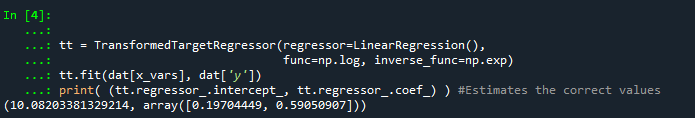But as we will see in a second, the exponentiated predictions are not so well behaved. To illustrate how the `WrongTrans` variable behaves, I show its distribution compared to the original `y` value. You can see that on average it is a much smaller estimate. Our sample values have a mean of 7.5 million, and the naive estimate here only has a mean of 4.6 million.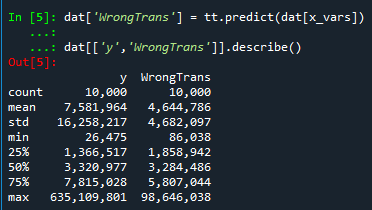Now here is a way to get an estimate of the mean value. In a nutshell, what you do is take the observed residuals, pretty much like that little table I did in the intro of this blog post, generate predictions given those residuals, and then back transform them and take the mean.

Although this example is using logged regression, I’ve made it pretty general. So if you used any box cox transformation instead of the logged (e.g. sklearns power_transform, it will work.

``````######################################################
# Duan's smearing, non-parametric approach via residuals

# Can make this general for any function inside of
# TransformedTargetRegressor
f = tt.get_params()['func']              #function
inv_f = tt.get_params()['inverse_func']  #and inverse function

# Non-parametric approach, approximate via residuals
# Using numpy broadcasting
log_pred = f(dat['WrongTrans'])
resids = f(dat['y']) - log_pred
resids = resids.values.reshape(1,n)
dp = inv_f(log_pred.values.reshape(n,1) + resids)
dat['DuanPreds'] = dp.mean(axis=1)

dat[['y','WrongTrans','DuanPreds']].describe()
######################################################``````

So you can see that the Duan Smeared predictions are looking better, at least the mean of the predictions is much closer to the original.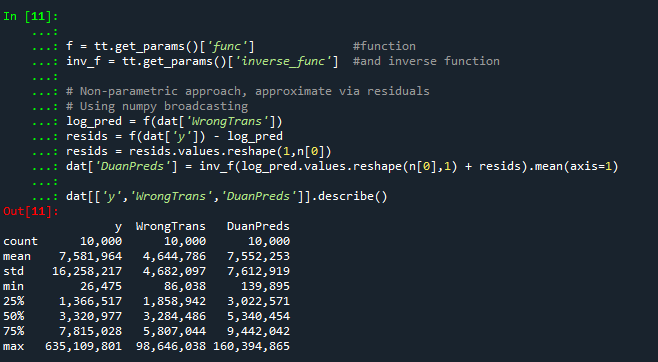I’ve intentionally done this example without using train/test, as we know the true answers. But in that case, you will want to use the residuals from the training dataset to apply this transformation to the test dataset.

So the residuals and the Duan smearing estimator do not need to be the same dimension. So for example if you have a big data application, you may want to do something like `resids = resids.sample(1000)` above.

Also another nice perk of this is you can use `dp` above to give you prediction intervals, so `np.quantile(dp,[0.025,0.975], axis=1).T` would give you a 95% prediction interval of the mean on the linear scale as well.

# Extra, Parametric Estimation

Another approach, which may make sense given the application, is instead of using the observed residuals to give a non-parametric estimate, you can estimate the distribution of the residuals, and then use that to make either an integral estimate of the Smeared estimate back on the original scale. Or in the case of the logged regression there is a closed form solution.

I show how to construct the integral estimator below, again trying to be more general. The integral approach will work for say any box-cox transformation.

``````######################################################
# Parametric approach, approximating residuals via normal

from scipy.stats import norm
from scipy.integrate import quad

# Look at the residuals again
resids = f(dat['y']) - f(tt.predict(dat[x_vars]))

# Check to make sure that the residuals are really close to normal
# Before doing this
resids.hist(bins=100)

# Fit to a normal distribution
loc, scale = norm.fit(resids)

# Define integral
def integrand(x,pred):
return norm.pdf(x, loc, scale)*inv_f(pred - x)

# Pred should be the logged prediction
# -50,50 should be changed if the residuals are scaled differently
def duan_param(pred):
return quad(integrand, -50, 50, args=(pred))

# This takes awhile to apply to the whole data frame!
dat['log_pred'] = f(tt.predict(dat[x_vars]))
sub_dat = dat.head(100).copy()
sub_dat['DuanParam'] = sub_dat['log_pred'].apply(duan_param)

# Can see that these are very similar to the non-parametric
print( sub_dat[['DuanPreds','DuanParam']].head(10) )``````

And you can see that this normal based approximation works just fine here, since by construction the model residuals are pretty well behaved in my simulation.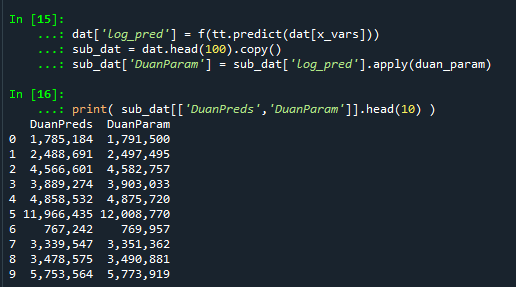It happens to be the case that there is a simpler estimate than the integral approach (which you can see in my notes takes awhile to estimate).

``````###########
# Easier way, but only applicable to log transform
# https://en.wikipedia.org/wiki/Smearing_retransformation
test_val = np.log(5000000)

# Integral approach
print( duan_param(test_val) )

# Approach for just log transformed
mult = np.exp(0.5*resids.var())
print( np.exp(test_val)*mult )
##########``````

So you can see the integral vs the closed form function are very close: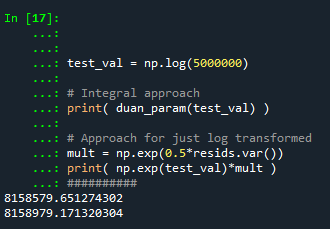The differences could be due to the the integral is simply an estimate (and you can see I did not do negative to positive infinity, but chopped it off, I do not know if there is a better function to estimate the integral or general approach here).

It wouldn’t surprise me if there are closed form solutions for box-cox transforms as well, but I am not familiar with them offhand. Again the integral approach (or the non-parametric approach) will work for whatever function you want. The function itself could be whatever crazy/discontinuous function you want. But this parametric Duan’s Smearing approach relies on the residuals being normally distributed. (I suppose you could use some other types of continuous distribution estimate if you have reason to, I have only seen normal distribution estimates though in practice.)

# Other Notes

While this focuses on regression, I do not think this will perform all that badly for other types of models (such as random forests or xgboost). But for forests it may make sense to simply pull out the individual tree estimates, back transform them, and get the mean of that backtransformed estimate. I have a different blog post that has a function showing how to scoop up the individual predictions from a random forest model.

It should also apply the same to any regression model with regularization. But if you want to do this, there are of course other alternative models you may consider that may be better suited towards your end goals of predictions on the linear/original scale.

For example, if you really want prediction intervals, it may make sense to not transform the data, and estimate a quantile regression model at the 5% and 95% quantiles. This would give you a 90% prediction interval.

Another approach is that it may make sense to use a different model, such as Poisson regression or negative binomial regression (or another generalized linear model in general). Even if your data are not integer counts, you can still use these models! (They just need to be 0 and above, no negative values.)

That Stata blog suggests to use Poisson and then robust standard errors, but that is a bad idea if you are really interested in predictions as well (see Gary Kings comment and linked paper). But you can just do negative binomial models in most cases then, and that is a better default than Poisson for many real world datasets.

# Geocoding the CMS NPI Registry (python)

So previously I wrote out creating service deserts. I have since found a nicer data source to use for this, the NPI CMS registry. This data file has over 6 million service providers across the US.

Here I will provide an example of using that data to geocode all the pharmacy’s in Texas, again using the census geocoding API and python.

# Chunking up the NPI database

So first, you can again download the entire NPI database from here. So I have already downloaded and unzipped that file, which contains a CSV for the January version, named `npidata_pfile_20050523-20210110.csv`. So as some upfront, here are the libraries I will be using, and I also set the directory to where my data is located.

``````###############################
import pandas as pd
import numpy as np
import censusgeocode as cg
import time
from datetime import datetime
import os
os.chdir(r'D:\HospitalData\NPPES_Data_Dissemination_January_2021')
###############################``````

The file is just a bit too big for me to fit in memory on my machine. On Windows, you can use `Get-Content npidata_pfile_20050523-20210110.csv | Measure-Object -Line` in powershell to get the line counts, or on Unix use `wc -l *.csv` for example. So I know the file is not quite 6.7 million rows.

So what I do here is create a function to read in the csv file in chunks, only select the columns and rows that I want, and then return that data frame. In the end, you need to search across all of the Taxonomy codes to pull out the type of service provider you want. So for community pharmacies, the code is `3336C0003X`, but it is not always in the first Taxonomy slot (some CVS’s have it in the second slot for example). You can see the big list of taxonomy codes here, so my criminology friends may say be interested in mental health or substance abuse service providers for other examples.

In addition to the taxonomy code, I always select organizations, not individuals (`Entity Type = 2`). And then I only select out pharmacies in Texas (although I bet you could fit all of the US pharmacies in memory pretty easily, maybe 60k in total?) Caveat emptor, I am not 100% sure how to use the deactivation codes properly in this database, as that data is always NaN for Texas pharmacies.

``````######################################################################
# Prepping the input data in chunks

keep_col = ['NPI','Entity Type Code','Provider Organization Name (Legal Business Name)',
'NPI Deactivation Reason Code','NPI Deactivation Date','NPI Reactivation Date',
'Provider First Line Business Practice Location Address',
'Provider Business Practice Location Address City Name',
'Provider Business Practice Location Address State Name',
'Provider Business Practice Location Address Postal Code']

taxon_codes = ['Healthcare Provider Taxonomy Code_' + str(i+1) for i in range(15)]
keep_col += taxon_codes
community_pharm = '3336C0003X'
npi_csv = 'npidata_pfile_20050523-20210110.csv' #Newer files will prob change the name

# This defines the rows I want
def sub_rows(data):
ec = data['Entity Type Code'] == "2"
st = data['Provider Business Practice Location Address State Name'] == 'TX'
ta = (data[taxon_codes] == community_pharm).any(axis=1)
#ac = data['NPI Deactivation Reason Code'].isna()
all_together = ec & st & ta #& ac
sub = data[all_together]
return sub

def csv_chunks(file,chunk_size,keep_cols,row_sub):
# First lets get the header and figure out the column indices
header_fields = list(pd.read_csv(npi_csv, nrows=1))
header_locs = [header_fields.index(i) for i in keep_cols]
# Now reading in a chunk of data
skip = 1
it_n = 0
sub_n = 0
ret_chunk = chunk_size
fin_li_dat = []
while ret_chunk == chunk_size:
file_chunk = pd.read_csv(file, usecols=header_locs, skiprows=skip,
nrows=chunk_size, names=header_fields, dtype='str')
sub_dat = row_sub(file_chunk)
fin_li_dat.append( sub_dat.copy() )
skip += chunk_size
it_n += 1
sub_n += sub_dat.shape
print(f'Grabbed iter {it_n} total sub n so far {sub_n}')
ret_chunk = file_chunk.shape
fin_dat = pd.concat(fin_li_dat, axis=0)
return fin_dat

# Takes about 3 minutes
print( datetime.now() )
pharm_tx = csv_chunks(npi_csv, chunk_size=1000000, keep_cols=keep_col, row_sub=sub_rows)
print( datetime.now() )

# No deactivated codes in all of Texas
print( pharm_tx['NPI Deactivation Reason Code'].value_counts() )
######################################################################``````

So this ends up returning not quite 6800 pharmacies in all of Texas.

# Geocoding using the census API

So first, the address data is pretty well formatted. But for those new to geocoding, if you have end parts of address strings like `Apt 21` or `Suite C`, those endings will typically throw geocoders off the mark. So in just a few minutes, I noted the different strings that marked the parts of the addresses I should chop off, and wrote a function to clean those up. Besides that I just limit the zip code to 5 digits, as that field is a mix of 5 and 9 digit zipcodes.

``````######################################################################
# Now prepping the data for geocoding

ph_tx = pharm_tx.drop(columns=taxon_codes).reset_index(drop=True)

#['Provider First Line Business Practice Location Address', 'Provider Business Practice Location Address City Name', 'Provider Business Practice Location Address State Name', 'Provider Business Practice Location Address Postal Code']

# I just looked through the files and saw that after these strings are not needed
end_str = [' STE', ' SUITE', ' BLDG', ' TOWER', ', #', ' UNIT',
' APT', ' BUILDING',',', '#']

def clean_add(address):
add_new = address.upper()
for su in end_str:
sf = address.find(su)
if sf > -1:
add_new = add_new[0:sf]
add_new = add_new.replace('.','')
add_new = add_new.strip()
return add_new

# Some examples
clean_add('5700 S GESSNER DR STE G')
clean_add('10701-B WEST BELFORT SUITE 170')
clean_add('100 EAST UNIVERSITY BLVD.')
clean_add('5800 BELLAIRE BLVD BLDG 1')
clean_add('2434 N I-35 # S')

ph_tx['Zip5'] = ph_tx['Provider Business Practice Location Address Postal Code'].str[0:5]
ph_tx['Address'] = ph_tx['Provider First Line Business Practice Location Address'].apply(clean_add)
ph_tx.rename(columns={'Provider Business Practice Location Address City Name':'City',
'Provider Business Practice Location Address State Name':'State2'},
inplace=True)
######################################################################``````

Next is my function to use the batch geocoding in the census api. Note the census api is a bit finicky – technically the census api says you can do batches of up to 5k rows, but I tend to get kicked off for higher values. So here I have a function that chunks it up into tinier batch portions and submits to the API. (A better function would cache intermediate results and wrap all that jazz in a `try` function.)

`````` ######################################################################
#This function breaks up the input data frame into chunks
#For the census geocoding api
def split_geo(df, add, city, state, zipcode, chunk_size=500):
df_new = df.copy()
df_new.reset_index(inplace=True)
splits = np.ceil( df.shape/chunk_size)
chunk_li = np.array_split(df_new['index'], splits)
res_li = []
pick_fi = []
for i,c in enumerate(chunk_li):
# Grab data, export to csv
sub_data = df_new.loc[c, ['index',add,city,state,zipcode]]
sub_data.to_csv('temp_geo.csv',header=False,index=False)
# Geo the results and turn back into df
print(f'Geocoding round {int(i)+1} of {int(splits)}, {datetime.now()}')
result = cg.addressbatch('temp_geo.csv') #should try/except?
# May want to dump the intermediate results
#pi_str = f'pickres_{int(i)}.p'
#pickle.dump( favorite_color, open( pi_str, "wb" ) )
#pick_fi.append(pi_str.copy())
names = list(result.keys())
res_zl = []
for r in result:
res_zl.append( list(r.values()) )
res_df = pd.DataFrame(res_zl, columns=names)
res_li.append( res_df.copy() )
time.sleep(10) #sleep 10 seconds to not get cutoff from request
final_df = pd.concat(res_li)
final_df.rename(columns={'id':'row'}, inplace=True)
final_df.reset_index(inplace=True, drop=True)
# Clean up csv file
os.remove('temp_geo.csv')
return final_df
######################################################################``````

And now we are onto the final stage, actually running the geocoding function, and piping the end results to a csv file. (Which you can see the final version here.)

``````######################################################################
# Geocoding the data in chunks

# Takes around 35 minutes
geo_pharm = split_geo(ph_tx, add='Address', city='City', state='State2', zipcode='Zip5', chunk_size=100)

#What is the geocoding hit rate?
print( geo_pharm['match'].value_counts() )
# Only around 65%

# Now merging back with the original data if you want
# Not quite sorted how I need them
geo_pharm['rowN'] = geo_pharm['row'].astype(int)
gp2 = geo_pharm.sort_values(by='rowN').reset_index(drop=True)

# Fields I want
kg = ['address','match','lat','lon']
kd = ['NPI',
'Provider Organization Name (Legal Business Name)',
'Provider First Line Business Practice Location Address',
'Address','City','State2','Zip5']

final_pharm = pd.concat( [ph_tx[kd], gp2[kg]], axis=1 )

final_pharm.to_csv('Pharmacies_Texas.csv',index=False)
######################################################################``````

Unfortunately the geocoding hit rate is pretty disappointing, only around 65% in this sample. So if I were using this for a project, I would likely do a round of geocoding using the Google API (which is a bit more unforgiving for varied addresses), or perhaps build my own openstreet map geocoder for the US. (Or in general if you don’t have too many to review, doing it interactively in ArcGIS is very nice as well if you have access to Arc.)

# Filled contour plot in python

I’ve been making a chart that looks similar to this for a few different projects at work, so figured a quick blog post to show the notes of it would be useful.

So people often talk about setting a decision threshold to turn a predicted probability into a binary yes/no decision. E.g. do I do some process to this observation if the probability is 20%, 30%, 60%, etc. If you can identify the costs and benefits of making particular decisions, you can set a simple threshold to make that decision. For example, say you are sending adverts in the mail for a product. If the person buys the product, your company makes \$50, and the advert only costs \$1 to send. In this framework, if you have a predictive model for the probability the advert will be successful, then your decision threshold will look like this:

``\$50*probability - \$1``

So in this case you need the predicted probability to be above 2% to have an expected positive return on the investment of sending the advert. So if you have a probability of 10% for 2000 customers, you would expect to make `2000 * (50*0.1 - 1) = 8000`. The probabilities you get from your predictive model can be thought of as in the long run averages. Any single advert may be a bust, but if your model is right and you send out a bunch, you should make this much money in the end. (If you have a vector of varying probabilities, in R code the estimated revenue will then look like `prob <- runif(2000,0,0.1); pover <- prob > 0.02; sum( (50*prob - 1)*pover )`.)

But many of the decisions I work with are not a single number in the benefits column. I am working with medical insurance claims data at HMS, and often determining models to audit those claims in some way. In this framework, it is more important to audit a high dollar claim than a lower dollar claim, even if the higher dollar value claim has a lower probability. So I have been making the subsequent filled contour plot I am going to show in the next section to illustrate this.

# python contour plot

The code snippet is small enough to just copy-paste entirely. First, I generate data over a regular grid to illustrate different claim amounts and then probabilities. Then I use `np.meshgrid` to get the data in the right shape for the contour plot. The revenue estimates are then simply the probability times the claims amount, minus some fixed (often labor to audit the claim) cost. After that is is just idiosyncratic matplotlib code to make a nice filled contour.

``````# Example of making a revenue contour plot
import matplotlib.pyplot as plt
from matplotlib.ticker import StrMethodFormatter
import numpy as np

n = 500 #how small grid cells are
prob = np.linspace(0,0.5,n)
dollar = np.linspace(0,10000,n)
#np.logspace(0,np.log10(10000),n) #if you want to do logged

# Generate grid
X, Y = np.meshgrid(prob, dollar)

# Example generating revenue
fixed = 200
Rev = (Y*X) - fixed

fig, ax = plt.subplots()
CS = ax.contourf(X, Y, Rev, cmap='RdPu')
clb = fig.colorbar(CS)
#clb.ax.set_xlabel('Revenue') #Abit too wide
clb.ax.set_title('dollar') #html does not like the dollar sign
ax.set_xlabel('Probability')
ax.set_ylabel('Claim Amount')
ax.yaxis.set_major_formatter(StrMethodFormatter('\${x:,.0f}'))
plt.title('Revenue Contours')
plt.xticks(np.arange(0,0.6,0.1))
plt.yticks(np.arange(0,11000,1000))
plt.annotate('Revenue subtracts \$200 of fixed labor costs',
(0,0), (0, -50),
xycoords='axes fraction',
textcoords='offset points', va='top')
#plt.savefig('RevContour.png',dpi=500,bbox_inches='tight')
plt.show()``````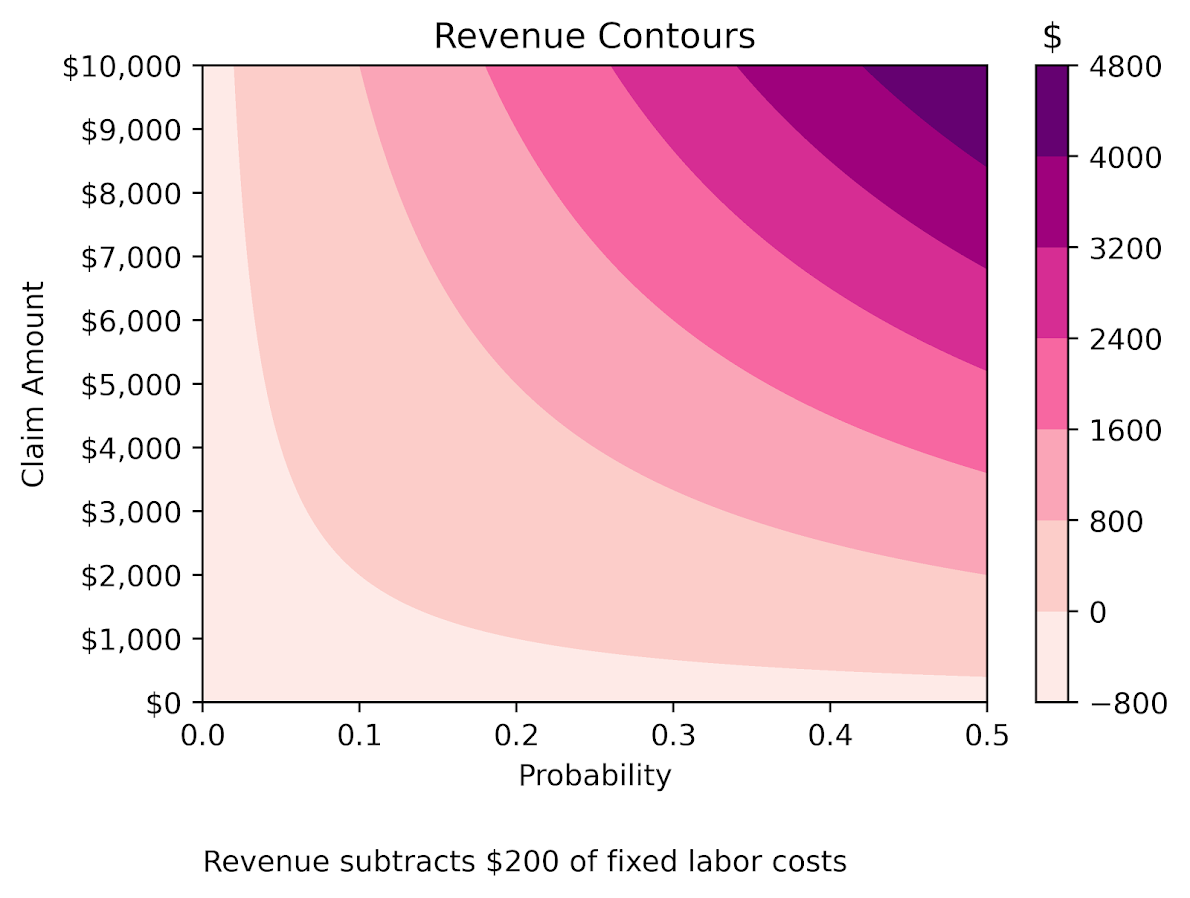The color bar does nice here out of the box. Next up in my personal learning will be how to manipulate color bars a bit more. Here I may want to either use a mask to not show negative expected returns, or a diverging color scheme (e.g. blue for negative returns).

# Buffers and hospital deserts with geopandas

Just a quick blog post today. As a bit of a side project at work I have been looking into medical service provider deserts. Most people simply use a geographic cutoff of say 1 mile (see Wissah et al., 2020 for example for Pharmacy deserts). Also for CJ folks, John Hipp has done some related work for parolees being nearby service providers (Hipp et al., 2009; 2011), measuring nearby as 2 miles.

So I wrote some code to calculate nice sequential buffer areas and dissolve them in geopandas. Files and code to showcase are here on GitHub. First, as an example dataset, I geocode (using the census geocoding API) CMS certified Home Healthcare facilities, so these are hospice facilities. To see a map of those facilities across the US, and you can click on the button to get info, go to here, CMS HOME FACILITY MAP. Below is a screenshot: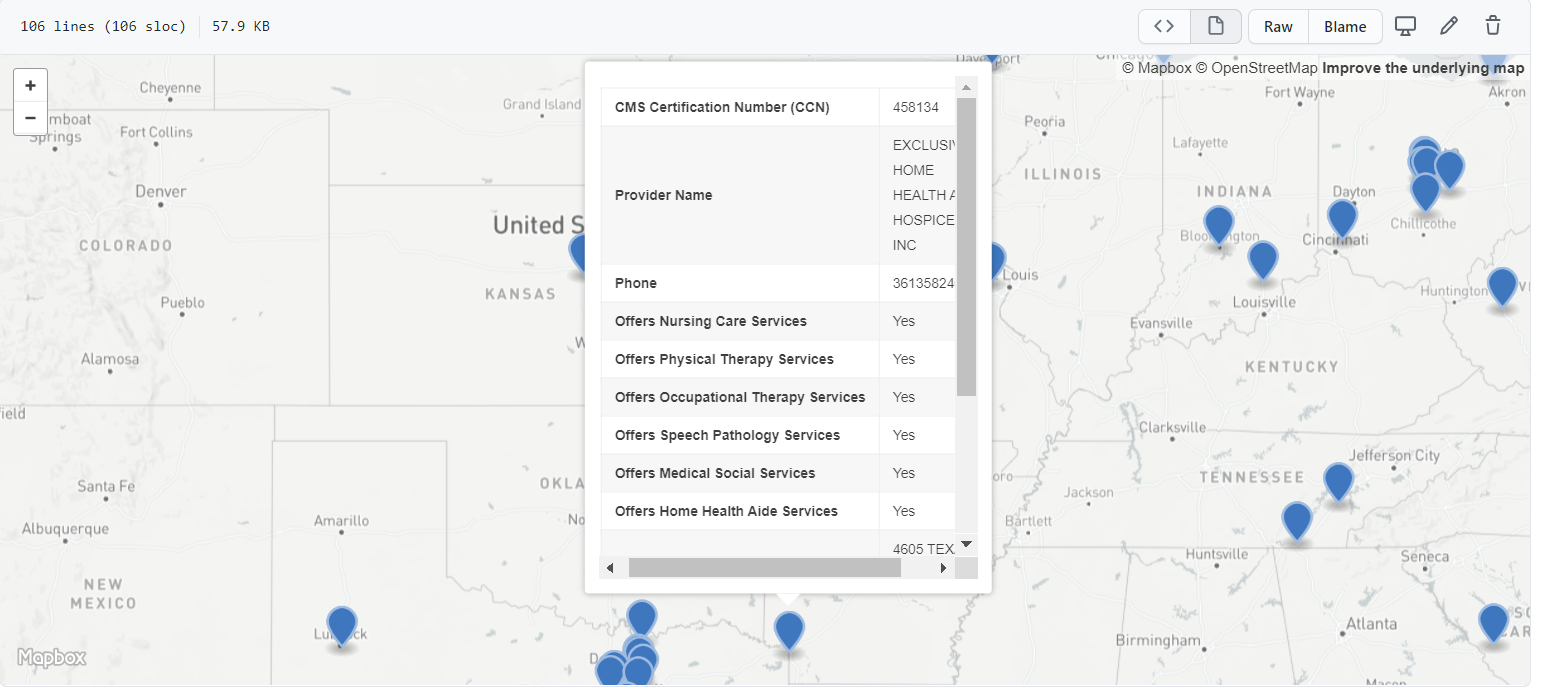Next I then generate sequential buffers in kilometers of 2, 4, 8, 16, and then the leftover (just for Texas). So you can then zoom in and darker areas are at a higher risk of not having a hospice facility nearby. HOSPICE DESERT MAP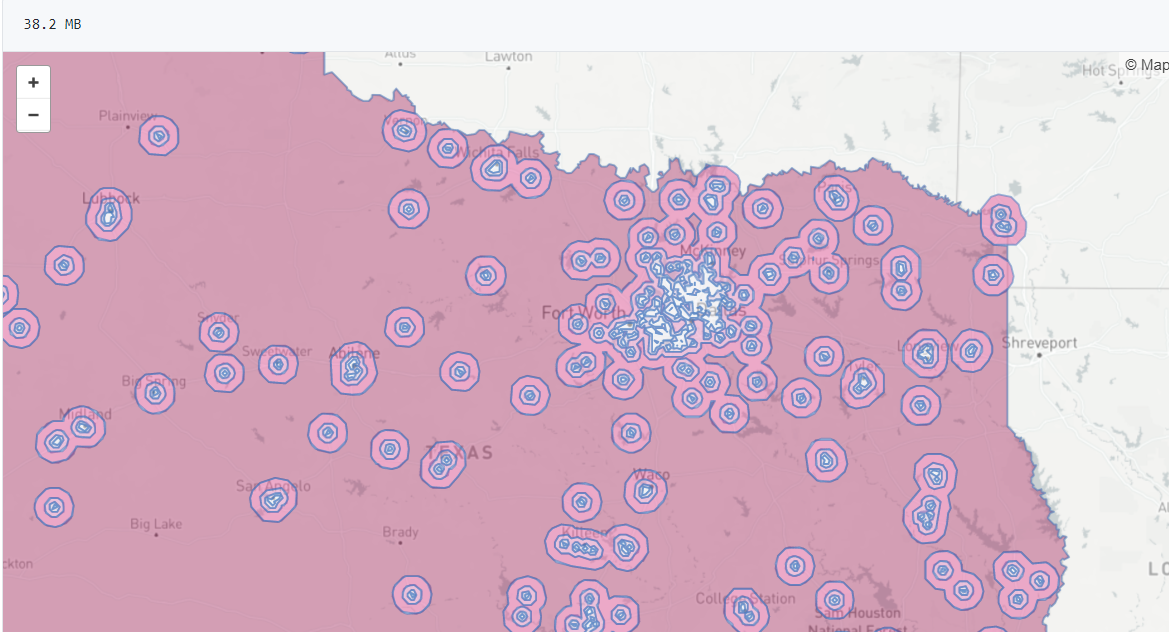Plotting some of these in Folium were giving me fits, so I will need to familiarize myself with that more in the future. The buffers for the full US as well were giving me trouble (these just for Texas result in fairly large files, surprised Github doesn’t yell at me for them being too big).

Going forward, what I want to do is instead of relying on a fixed function of distance, is to fit a model to identify individuals probability of going to the doctor based on distance. So instead of just saying 1+ mile and you are at high risk, fit a function that defines that distance based on behavioral data (maybe using insurance claims). Also I think the distances matter quite a bit for urban/rural and car/no-car. So rural folks traveling a mile is not a big deal, since you need a car to really do anything in rural areas. But for folks in the city relying on public transportation going a mile or two is a bigger deal.

The model then would be similar to the work I did with Gio on gunshot death risk (Circo & Wheeler, 2020), although I imagine the model would spatially vary (so maybe geographically weighted regression may work out well).

# The WDD test with different pre/post time periods

Eric Piza asked the other day if my and Jerry’s WDD test can be used when the pre/post time periods are different. The answer is yes out of the box, the identification strategy does not rely on equality of time periods. So for example, say we had two years pre and one year post data, and the crime counts in treated/control looked like this:

``````         Pre  Post
Treated   80    20
Control  100    50``````

So then our difference-in-difference Poisson estimate of the treatment effect would be:

``(20 - 80) - (50 - 100) =  -10``

What the parallel trends assumption means here is that since you saw a decrease in 50 crimes in the control area, you would expect a decrease of 50 crimes in the treated area as well. The variance of this estimate is then `20 + 80 + 50 + 100 = 250`, and so the standard error is `sqrt(250) ~ 15.8`. So this is not a statistically significant effect.

It is hard to interpret this effect size though, since it is not a standard unit of time comparison. Also the variance of the estimate will be larger if you have a longer pre time period, which is the opposite of what you want. We can actually amend the statistic though to be a per-unit-time comparison, which will reduce the variance of the estimate. It ends up being similar to my prior post on adding Harm Weights to the WDD, you can’t just pipe in the per unit time estimates in the spreadsheet I shared, but I will show here how to incorporate them into the estimator (and share some python code to show the estimator behaves as expected in simulations).

So again with a pre-time period of 2 years, and post of 1 year, we could do the prior table as per year estimates.

``````         Pre  Post
Treated   40    20
Control   50    50``````

And here our estimate of the crime reduction effect is different:

``(20 - 40) - (50 - 50) =  -20``

So with a Poisson variable with a mean of 100, the variance of that variable is also 100. So here we are dividing that 100 by a constant 2 – this changes the variance to 100/(2^2). (`Var(X*a) = a^2*Var(X)` where `X` is a random variable and `a` is a constant.) The post variables are simply divided by one, so does not change their variance. So to carry this forward to our standard error estimate, we would calculate:

``20/1 + 40/4 + 50/1 + 50/4 = 92.5``

So you can see that our variance estimate here is much smaller, and that the standard error is `sqrt(92.5) ~ 9.6`. So here the reduction is right on the border of a statistically significant reduction in crimes. A 95% confidence interval would be `-20 +/- 2*9.6 ~ [-1, -39]`. Here the WDD estimate is easier to interpret as well, and that confidence interval corresponds to a per year estimate reduction of somewhere between 1 and 39 crimes.

Below I share some python code to conduct simulations similar to the original WDD paper. This code will then establish the estimator has the null distribution as expected (when there are no changes it really is a standard normal distribution) and that the confidence intervals have coverage like you would expect.

# Python Simulation Code

For set up, I import the libraries I need (stat distributions, numpy and pandas). I am not going to go into detail into the functions, but it allows you to generate simulated distributions in various ways to conduct analysis of the properties of my time weighted estimator I have specified above.

``````'''
WDD Simulation with differing time periods
Andy Wheeler
'''

import pandas as pd
import numpy as np
from scipy.stats import norm
from scipy.stats import poisson
from scipy.stats import uniform

#This works for the scipy functions
np.random.seed(seed=10)

# A function to generate the WDD estimate for simulated data
def wdd_sim(treat0,treat1,cont0,cont1,pre,post):
tr_cr_0 = poisson.rvs(mu = treat0, size=int(pre)).sum()
co_cr_0 = poisson.rvs(mu = cont0, size=int(pre)).sum()
tr_cr_1 = poisson.rvs(mu = treat1, size=int(post)).sum()
co_cr_1 = poisson.rvs(mu = cont1, size=int(post)).sum()
est = ( tr_cr_1/post - tr_cr_0/pre ) - ( co_cr_1/post - co_cr_0/pre )
post2 = (1/post)**2
pre2 = (1/pre)**2
var_est = tr_cr_0*pre2 + tr_cr_1*post2 + co_cr_0*pre2 + co_cr_1*post2
true_val = ( treat1 - treat0 ) - ( cont1 - cont0 )
z_score = est / np.sqrt(var_est)
return (est, var_est, true_val, z_score)

def make_data(n, treat0, treat1, cont0, cont1, pre, post):
base = pd.DataFrame( range(n), columns=['index'])
base['treat0'] = treat0
if treat1 is not None:
base['treat1'] = treat1
else:
base['treat1'] = base['treat0']
if cont0 is not None:
base['cont0'] = cont0
else:
base['cont0'] = base['treat0']
if cont1 is not None:
base['cont1'] = cont1
else:
base['cont1'] = base['cont0']
base.drop(columns='index',inplace=True)
base['pre'] = pre
base['post'] = post
sim_vals = base.apply(lambda x: wdd_sim(**x), axis=1, result_type='expand')
sim_vals.columns = ['est','var_est','true_val','z_score']
return pd.concat([base,sim_vals], axis=1)``````

So for a first example, this code generates treatment/control areas with a Poisson mean of 5 in both the pre/post time periods. But, the pre time period is 4 units of time, and the post time period is only 1 unit. So this means there is no change, and the Z score estimator should on average have a 0 estimate and a standard deviation of 1. I do 10,000 simulations to keep it going a bit faster, but you can up that if you want.

``````# No change, with baseline of 5 crimes per unit time
sim_dat = make_data(10000, 5, 5, 5, 5, 4, 1)
sim_dat['z_score'].describe()``````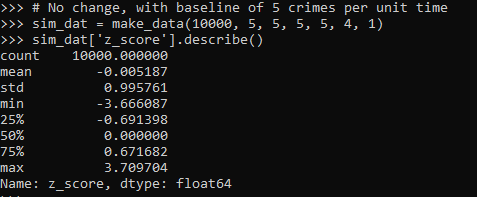So here we can see these 10k simulated Poisson data have a mean z-score of 0 and a standard deviation of 1, right like we expected.

So I haven’t extensively tested, but if you have average crime counts well under 5, I would be a bit hesitant to use this estimator. (So you either need larger area aggregations or larger time aggregations.) Although you could do simulations on your own to see how it holds up.

The way I wrote the functions you can also pass in random variables as well, so here is an example with again no change, but the baseline varies uniformily from 5 to 100. And here also the pre time periods are 6, and the post time period is again just 1.

``````# Can pass in random functions instead of constant values
sim_n = 10000
tf = uniform.rvs(loc=5, scale=100, size=sim_n)

sim_dat2 = make_data(sim_n, tf, None, None, None, 6, 1)
sim_dat2.head()
sim_dat2['z_score'].describe()``````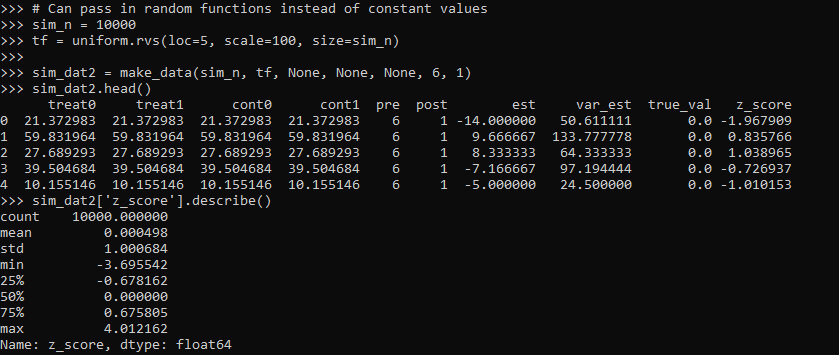So you can see the base simulated dataset pre/post always has the same means, but instead of being a set of constant 5’s, it changes for each row (simulation) in the dataset. And again the null distribution is right on the money with a mean of 0 and standard deviation of 1.

So those are examples of the null distribution of no changes in the time weighted estimator. This establishes that the false positive alpha rates are as you would expect. E.g. if you use the usual p-value < 0.05, if the differences are really 0 you only have a false positive reject the null 5 times out of 100.

But we also want to establish that when there is a difference, the estimator is not biased and that the variance estimates are correct. For the later part looking at the coverage rates of the confidence intervals is one way to do that. So here I show that with my hypothetical example in the intro part of this blog, the 95% and 90% confidence interval coverage rates are exactly as they should be. And the z-score estimate is right about where it should be as well.

``````# Lets look at the coverage rate for a decline from 40 to 20
def cover(data, ci=0.95):
mult = (1 - ci)/2
nv = norm.ppf(1 - mult)
dif = nv*np.sqrt( data['var_est'] )
low = data['est'] - dif
high = data['est'] + dif
cover = ( data['true_val'] > low) & ( data['true_val'] < high )
return cover

sim_dat3 = make_data(sim_n, 40, 20, 50, 50, 2, 1)
sim_dat3.head()

# This should be centered on 2
sim_dat3['z_score'].describe()

# Should be ~ 0.9
co_90 = cover(sim_dat3, ci=0.9)
co_90.mean()

# Should be ~ 0.95
co_95 = cover(sim_dat3, ci=0.95)
co_95.mean()``````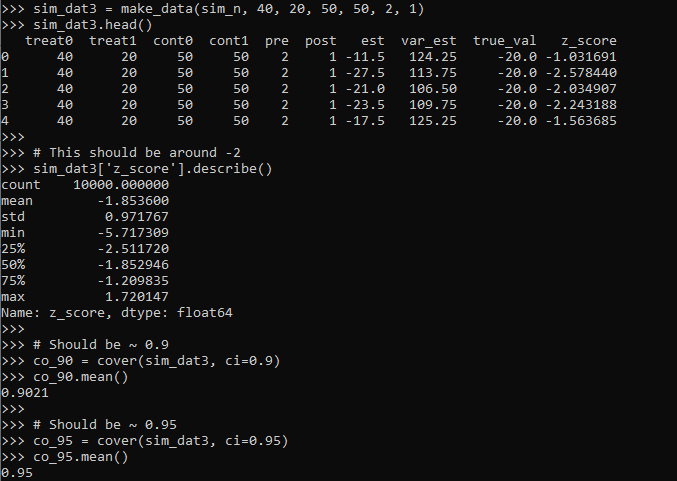So you can see the coverage is right on the money. The estimator is slightly biased downward in this simulation (should get a z-score on average around -2, but here the mean is -1.85). But it is good enough IMO to not worry about much in this situation.

Again, the original estimator without weighted for time is fine, if we do the same motions without doing weighting for different time periods, the coverage is still all fine and dandy.

``````# Note you can do the same coverage estimate without time weighted
sim_dat4 = make_data(sim_n, 80, 20, 100, 50, 1, 1)
sim_dat4.head()

# This should be around -0.6
sim_dat4['z_score'].describe()

co_90w = cover(sim_dat4, ci=0.9)
co_90w.mean()

co_95w = cover(sim_dat4, ci=0.95)
co_95w.mean()``````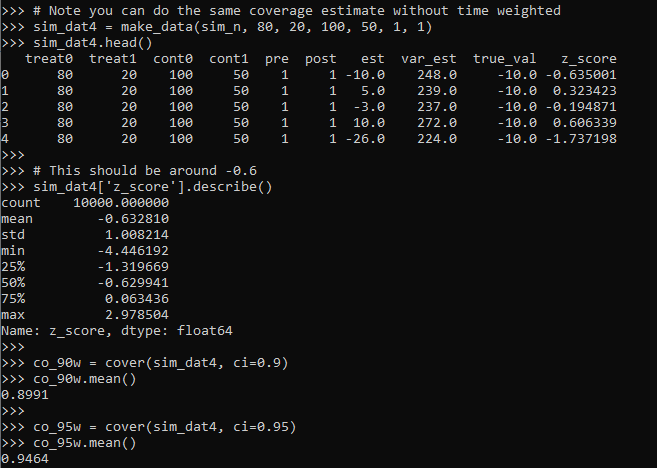So you can see again coverage is right on the money, and the z-score estimator actually has less bias than the time weighted one, it is right on the money as expected.

So why would you prefer the time weighted estimator if it shows more bias? It is because it has a lower variance, this code shows the length of the confidence intervals in the simulations.

``````# Does it make a difference?
def len_ci(data, ci=0.95):
mult = (1 - ci)/2
nv = norm.ppf(1 - mult)
dif = nv*np.sqrt( data['var_est'] )
low = data['est'] - dif
high = data['est'] + dif
return high - low

len4 = len_ci(sim_dat4)
len4.describe()

len3 = len_ci(sim_dat3)
len3.describe()``````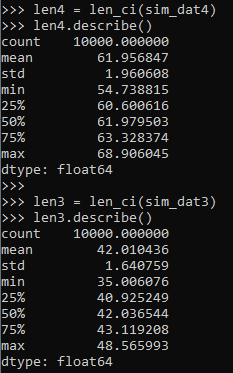So you can see here that the non-time weighted estimator tends to have a confidence interval with a length of 62, whereas the time weighted estimator has a confidence interval on average of 42.

So above establishes that the time weighted estimator behaves as you would expect. You can also use this code to conduct some potential power analyses. So for the time weighted estimator we show, even though the reduction is around 50% in the treated area (going from 40 to 20), the power is not great, around 60%.

``````# Example power analyses, ONE TAILED
def reject_rate(data, alpha=0.05):
p_vals = norm.cdf(data['z_score'])
return p_vals < alpha

r3 = reject_rate(sim_dat3)
r3.mean()``````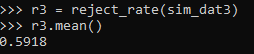So this means if you did this experiment in real life and it was that effective, you would still fail to reject the null of no differences 2/5 times.

But what if we say we will get more historical data? So 4 years back instead of just 2? How does that impact our power estimates?

``````# How about with more historical data
sim_dat5 = make_data(sim_n, 40, 20, 50, 50, 4, 1)
r5 = reject_rate(sim_dat5)
r5.mean()``````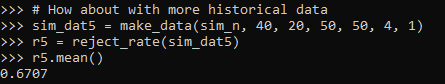The power goes up by alittle, to 0.67. The same is true if we up the post period to 4 time periods instead of 1:

``````# How about with more post data
sim_dat6 = make_data(sim_n, 40, 20, 50, 50, 4, 4)
r6 = reject_rate(sim_dat6)
r6.mean()``````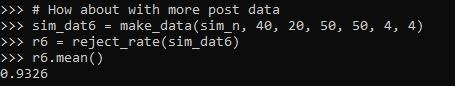So now in this example you have an over 90% power to detect a crime reduction, going from 40 to 20 per time period (where the control has an average of 50 crimes per time period), if you have 4 pre time periods and 4 post time periods.

# Future Stuff

So a few caveats with this. For one, you may think that since dividing per time period reduces the variance, why not divide by smaller time slivers. So instead of one year, why not divide by 365 days?

I have not studied extensively this property of the estimator. So I cannot say how it behaves with more/less time aggregation into smaller Poisson estimates. You will need to take that on yourself if you want to examine very fine time units and very small Poisson counts per unit time. Again I think a baseline rule of thumb that they should not be lower the 5 counts per unit time is the best advice I can give without doing simulations for your exact circumstances.

A second part is that with longer time periods comes the risk that the control areas are not as good. This is a problem intrinsic to synthetic control analysis as well (that I don’t believe anyone has a particular answer to). And I don’t have an answer either.

For the pre-time period, you can check the parallel trends assumption by simply plotting the two time series, they should be close to in step with one another. So that is not a big deal. But with the post time period, I think if you monitor long enough they will eventually depart from one another.

So I think it is best to set up a time period at the start you have committed to doing the experiment. And you can use the power analysis simulations like I showed to help you figure out that period. But it may be possible to extend this WDD estimate to continuously monitor an intervention (see here for example).

# A changepoint logistic model in pystan

So the other day I showed how to use the mcp library in R to estimate a changepoint model with an unknown changepoint location. I was able to get a similar model to work in pystan, although it ends up being slower in practice than the mcp library (which uses JAGS under the hood). It also limits the changepoints to a specific grid of values. So offhand there isn’t a specific reason to prefer this approach to the R mcp library, but I post here to show my work. Also I illustrate that with this particular model, using 1000 simulated observations.

To be clear what this model is, instead of the many time series examples floating around about changepoints (like the one in the Stan guide), we have a model with a particular continuous independent variable x, and we are predicting the probability of something based on that x variable. It is not that different, but many of those time series examples the universe to check for changepoints is obvious, only the observed time series locations. But here we have a continuous input (distance a crime event is from a CCTV camera), but we can only check a finite number of locations. It ends up being closer in spirit to this recent post by Keith Goldfield.

So in some quick and dirty text math, here `c` is the changepoint location and `l` is the logit function:

``````l(Prob[y]) = intercept + b1*x; if x <= c
l(Prob[y]) = intercept + b1*x + b2*(x - c); if x > c``````

This model can be expanded however you want – such as other covariates that do not change with the changepoint. But for this simple simulation I am just looking at the one running variable x and the binary outcome y.

# Python Code

So first, I load up the libraries I will be using, then I simulate some data. Here the changepoint is located at 0.42 for the x variable, and in the `ylogit` line you can see the underlying logistic regression equation.

``````#################################
# Libraries I am using
import pystan
import numpy as np
import pandas as pd
import statsmodels.api as sm
#################################

#################################
# Creating simulated data
np.random.seed(10)
total_cases = 1000 #30000
x = np.random.uniform(size=total_cases) #[total_cases,1]
change = 0.42
xdif = (x - change)*(x > change)
ylogit = 1.1 + -4.3*x + 2.4*xdif
yprob = 1/(1 + np.exp(-ylogit))
ybin = np.random.binomial(1,yprob)
#################################``````

When testing out these models, one mistake I made was thinking offhand that 1,000 observations should be plenty. (Easier to run more draws with a smaller dataset.) When I had smaller effect sizes, the logistic coefficients could be pretty badly biased. So I started as a check estimating the logistic model inputting the correct changepoint location. Those biased estimates are pretty much the best case scenario you could hope for in the subsequent MCMC models. So here is an example fitting a logit model inputting the correct location for the changepoint.

``````#################################
#Statsmodel code to get
#The coefficient estimates
#And standard errors for the sims
con = *len(x)
xcomb = pd.DataFrame(zip(con,list(x),list(xdif)),columns=['const','x','xdif'])
log_reg = sm.Logit(ybin, xcomb).fit()
print(log_reg.summary())
#################################``````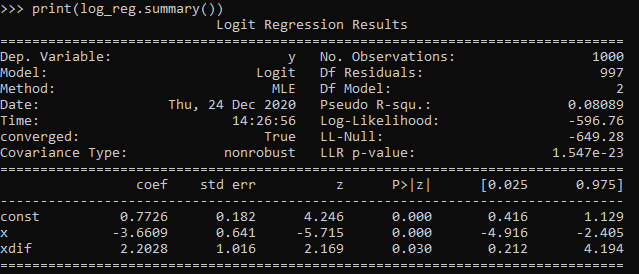So you can see that my coefficient estimates and the frequentist standard errors are pretty large even with 1,000 samples. So I shouldn’t expect my later MCMC model to have any smaller credible intervals than above.

So here is the Stan model. I am using pystan here, but of course it would be the same text file if you wanted to fit the model using R. (Just compiles C++ code under the hood.) Of only real note is that I show how to use the softmax function to estimate the actual mean location of the changepoint. Note that that mean summary though only makes sense if you make your grid of changepoint locations regular and fairly fine. (So if you said a changepoint could be at 0.1, 0.36, and 0.87, taking a weighted mean of those three locations doesn’t make sense.)

``````#################################
#Stan model
changepoint_stan = """
data {
int<lower=1> N;
vector[N] x;
int<lower=0,upper=1> y[N];
int<lower=1> Samp_Points;
vector[Samp_Points] change;
}
transformed data {
real log_unif;
log_unif = -log(Samp_Points);
}
parameters {
real intercept;
real b_x;
real b_c;
}
transformed parameters {
vector[Samp_Points] lp;
real before;
real after;
lp = rep_vector(log_unif, Samp_Points);
for (c in 1:Samp_Points){
for (n in 1:N){
before = intercept + b_x*x[n];
after = before + b_c*(x[n] - change[c]);
lp[c] = lp[c] + bernoulli_logit_lpmf(y[n] | x[n] < change[c] ? before : after );
}
}
}
model {
intercept ~ normal(0.0, 10.0);
b_x ~ normal(0.0, 10.0);
b_c ~ normal(0.0, 10.0);
target += log_sum_exp(lp);
}
generated quantities {
vector[Samp_Points] prob_point;
real change_loc;
prob_point = softmax(lp);
change_loc = sum( prob_point .* change );
}
"""
#################################``````

And finally I show how to prepare the data for pystan (as a dictionary), compile the model, and then draw a ton of samples. I generate a regular grid of 0.01 intervals from 0.03 to 0.97 (can’t have a changepoint outside of the x data locations, which I drew as a random uniform 0,l). Note the more typical default of 1000 tended to not converge, the effective number of samples is quite small for that many. So 5k to 10k samples in my experiments tended to converge. Note that this is not real fast either, took about 40 minutes on my machine (the Stan guesstimates for time were always pretty good ballpark figures).

``````#################################
# Prepping data and fitting the model

stan_dat = {'N': ybin.shape}
stan_dat['change'] = np.linspace(0.03,0.97,95) #[0.1, 0.2, 0.3, 0.4, 0.5, 0.6, 0.7, 0.8, 0.9]
stan_dat['Samp_Points'] = len(stan_dat['change'])
stan_dat['x'] = x
stan_dat['y'] = ybin

sm = pystan.StanModel(model_code=changepoint_stan)
#My examples needed more like 10,000 iterations
#effective sample size very low, took about 40 minutes on my machine
fit = sm.sampling(data=stan_dat, iter=5000,
warmup=500, chains=4, verbose=True)
#Prints some results at the terminal!
print(fit.stansummary(pars=['change_loc','intercept','b_x','b_c']))
#################################``````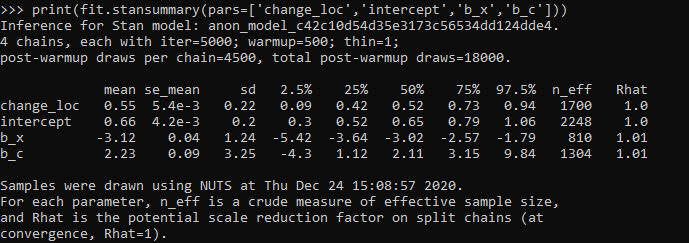So you can see the results – the credible intervals for the intercept and regression coefficient before the change point are not bad, just slightly larger than the logistic model. The credible interval for the changepoint location and the changepoint effect different are quite uncertain though. The changepoint location covers almost the whole interval I examined. It may be better to plot the individual probabilities, like Goldfield did in his post, as opposed to summarized a mean location for the distribution (which is discrete in the end based on your grid of locations you look at).

So that at least gives a partial warning that you need quite big data samples to effectively identify the changepoint location, at least for this Stan model as I have shown. I haven’t run it on my 26k actual data sample, as it will end up taking my computer around 30 hours to crunch out 10k draws with 4 chains. Next up I rather see if I can get a similar model working in pyro, as my GPU on my personal machine I think will be faster than the C++ code here. (There are probably smarter ways to vectorize the Stan model as well.)

# Confidence intervals around proportions

So you probably learned about confidence intervals around means in your introductory statistics class. For a refresher, a confidence interval covers a particular statistic at a pre-specified rate. So if I generate 100 90% intervals around a mean, I expect that those confidence intervals would cover the true underlying mean around 90 times out of those 100. So it is a statement about the procedure overall – not any individual test.

This repeated coverage property is often not exactly what we want in statistics. But, I often find examining confidence intervals around samples to be an informative way to quantify uncertainty in estimates. For example, I have a machine learning model serving up predictions to a subsequent auditing process. I expect this to maintain a hit rate above 20%. The past week I only had a hit rate of 30/200 (15%), should I be worried? Probably not, a 95% confidence interval around that proportion is 10% to 21%.

Proportions come up so often that intro stats courses should probably talk more extensively about generating confidence intervals around them. There are many different confidence intervals for proportions, Wikipedia lists 7 different options!

I prefer where possible to use the Clopper-Pearson intervals by default. I will show an examples of generating Clopper-Pearson intervals in Excel and Python. But, another situation I have come across is I want to do these intervals entirely in SQL. For that situation, I will show how to use Agresti–Coull intervals.

# Excel Clopper-Pearson

In Excel, if the `A` column contains the numerator, the `B` column contains the denominator, and if `G1` has the alpha level, this brutish formula gets you the lower bound of your confidence interval;

``=IF(A2=0, 0, BETA.INV(\$G\$1/2, A2, B2-A2+1))``

A here is your upper bound;

``=IF(A2=B2, 1, BETA.INV(1-\$G\$1/2, A2+1, B2-A2))``

And here is a screenshot of the filled in results: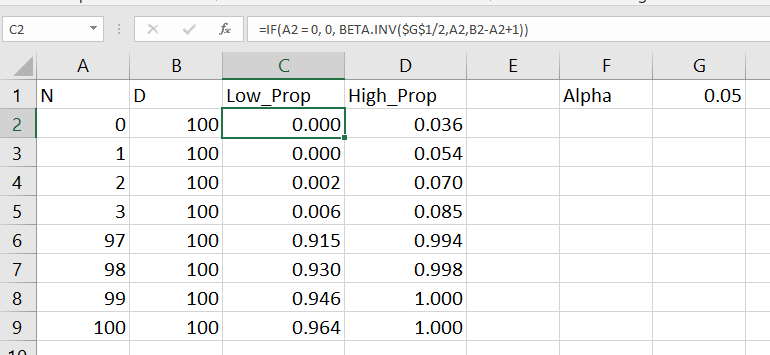Note for my criminology friends, you can use this for very extreme proportions as well. So say you had a homicide rate of 10 per 100,000, where the observed sample was 30 homicides in a city of 300,000. You can generate a binomial confidence interval around the proportion and then translate back to the rate per 100,000. So in that scenario, it results in a 95% confidence interval of a homicide rate of 6.7 to 14.3.

This is actually the reason I like defaulting to Clopper-Pearson. The other approximations can fail very badly for extreme tail events like this.

# Python Clopper-Pearson

Here is a simple function in python to return the Clopper-Pearson intervals. This works for vectorized inputs as well (e.g. numpy arrays or pandas series).

``````import numpy as np
from scipy.stats import beta

def binom_int(num,den, confint=0.95):
quant = (1 - confint)/ 2.
low = beta.ppf(quant, num, den - num + 1)
high = beta.ppf(1 - quant, num + 1, den - num)
return (np.nan_to_num(low), np.where(np.isnan(high), 1, high))``````

And here is an example use:

``````hits = np.array([0, 1, 2, 3, 97, 98, 99, 100])
tries = np.array(*len(hits))
lowCI, highCI = binom_int(hits, tries)``````

Check out my prior blog post on making smoothed scatterplots on how to plot those proportion spikes in matplotlib.

# SQL Agresti–Coull

So as I mentioned previously, I prefer the Clopper-Pearson intervals. This however relies on the availability of a function for the inverse beta distribution. One common situation is I just have all my tables in SQL, and I want to make a dashboard connected to a view of my tables. So the proportion of some event broken downs by days/weeks/months etc.

In that case exporting the data to python and re-uploading to the database can be a bit of a hassle, whereas creating a view is much less work. So here is an example query to calculate the proportion intervals entirely in SQL. So the initial table is a micro level table of events with 0/1 for a particular group. (This screenshot is for Access, but this should work in various databases.)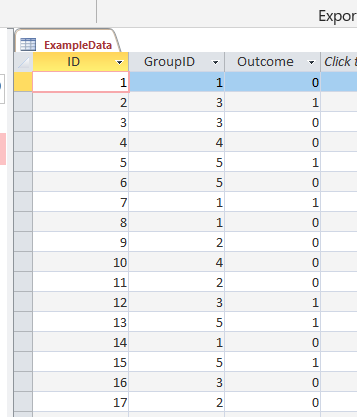And then it is a groupby to get the original numerator, denominator, and proportion. Then a few rows calculating the adjusted proportion (add 2 to the numerator and 2*2 to the denominator), then finally this can still produce lower than 0 and higher than 1 intervals, so I cap those off.

``````/* This is for Access, for others may want to use SQRT() instead of SQR()
Also may want to use CASE WHEN instead of IIF */
SELECT
GroupID,
SUM(Outcome) AS Num,
COUNT(Outcome) AS Den,
Num/Den AS Prop,
Num + 2 AS nadj,
Den + 2*2 as dadj,
nadj/dadj as padj,
2*SQR((padj/nadj)*(1 - padj)) AS zadj,
IIF( padj < zadj, 0, padj - zadj) AS LowCI,
IIF( (1 - padj) < zadj, 1, padj + zadj) AS HighCI
FROM ExampleData
GROUP BY GroupID;``````

This produces a 95% confidence interval for the final two columns. If you wanted to generate say a 99% confidence interval, you would replace the 2’s in the above table with `2.6`. (In R you can do `qnorm(1 - a/2)`, where `a` is `1 - confidence_level`, to figure out this constant.)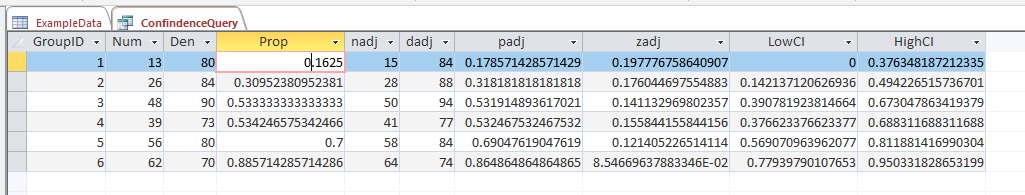# What you shouldn’t use these intervals for

While I believe many applications of dashboards are well suited to including confidence intervals, confidence intervals (like p-values) are apt to be misinterpreted. One common one is that for a single 95% confidence interval, that does not mean the interval covers the true estimate with a 95% probability. This is an inference for an individual sample that is not possible in frequentist statistics – that summary would be akin to a posterior credible interval in Bayesian statistics. Confidence intervals are about the procedure, if we do this procedure over and over again, in the long run it will cover the true statistic (which we do not observe for any individual sample), according to the level we set.

Another common mistake with confidence intervals is when comparing two different intervals, them overlapping is sometimes interpreted as no difference. But this is a very conservative test (e.g. will fail to reject the null of no differences too often).

So say we were monitoring a process over time, and in October the process was 20% (40/200) and in November it was 28% (168/600). October’s confidence interval is 15% to 26%, and November’s confidence interval is 24% to 32%. So since those intervals overlap, we should conclude there are no differences correct? Not exactly, if we do a direct test for the differences in proportions (akin to a t-test of mean differences), we get a confidence interval of the difference as -14% to -1% (in R `prop.test(c(40,168), c(200,600))`). So in that direct hypothesis test, we would conclude October’s percent is lower than Novembers percent.

Geoff Cumming suggests that when going from individual confidence intervals to comparisons between groups, one confidence interval needs to cover the point estimate for the other group to conclude the two groups are different.

But that being said, I believe many dashboards would be improved if incorporating such confidence intervals. So although they may not always provide the test of interest, they are a good way to prevent yourself from over-interpreting noisy trends in smaller samples. In the case of comparing two intervals, for most situations I deal with, being conservative in saying this process is not showing differences is a better approach than worrying about minor fluctuations (although just depends on the use case whether that default behavior makes sense.)

So please, when reporting proportions with small samples, provide a confidence interval around those proportions!

# Outliers in Distributions

If you google ‘outlier’, all of the results that come up are in terms of individual observation outliers. So if you have a set of transaction data that is `10, 20, 30, 8000`, the singlet observation `8000` is an outlier. But for many situations with transaction data, you don’t want to examine individual outlier incidents, but look for systematic patterns. For example, if I am looking at healthcare insurance claims for my work, a single claim that is \$100,000 is actually not that rare. But if we have a hospital that has mostly \$100,000 claims for a specific treatment, whereas another with similar cases has a range of \$50,000 to \$100,000, that may signal there is some funny business going on.

There is no singular way to examine outliers in distribution. A plain old t-test of mean differences may make sense for some situations. But a generally more useful way IMO to think about the problem is to examine the distribution of the outcome in CDF space, as opposed to looking at particular moments of the distribution. A t-test basically only looks at the differences in means for the distributions, whereas examining the CDF we are looking for weird patterns at any point in the distribution.

Here is an example of comparing the cost of hospital stays (per length of stay), for a hospital compared to all others from the same datasource (details on the data in a sec). The way to read this graph is that at 10^3 (so \$1000 per day claims) for facility 1458, we have around 20% of the claims data are below this value. For the rest of the hospital data, a larger proportion of claims are under a thousand dollars, more like 25%. Since the red line is always below the black line, it also means that the claims at this hospital are pretty much always larger than the claims at all the other hospitals.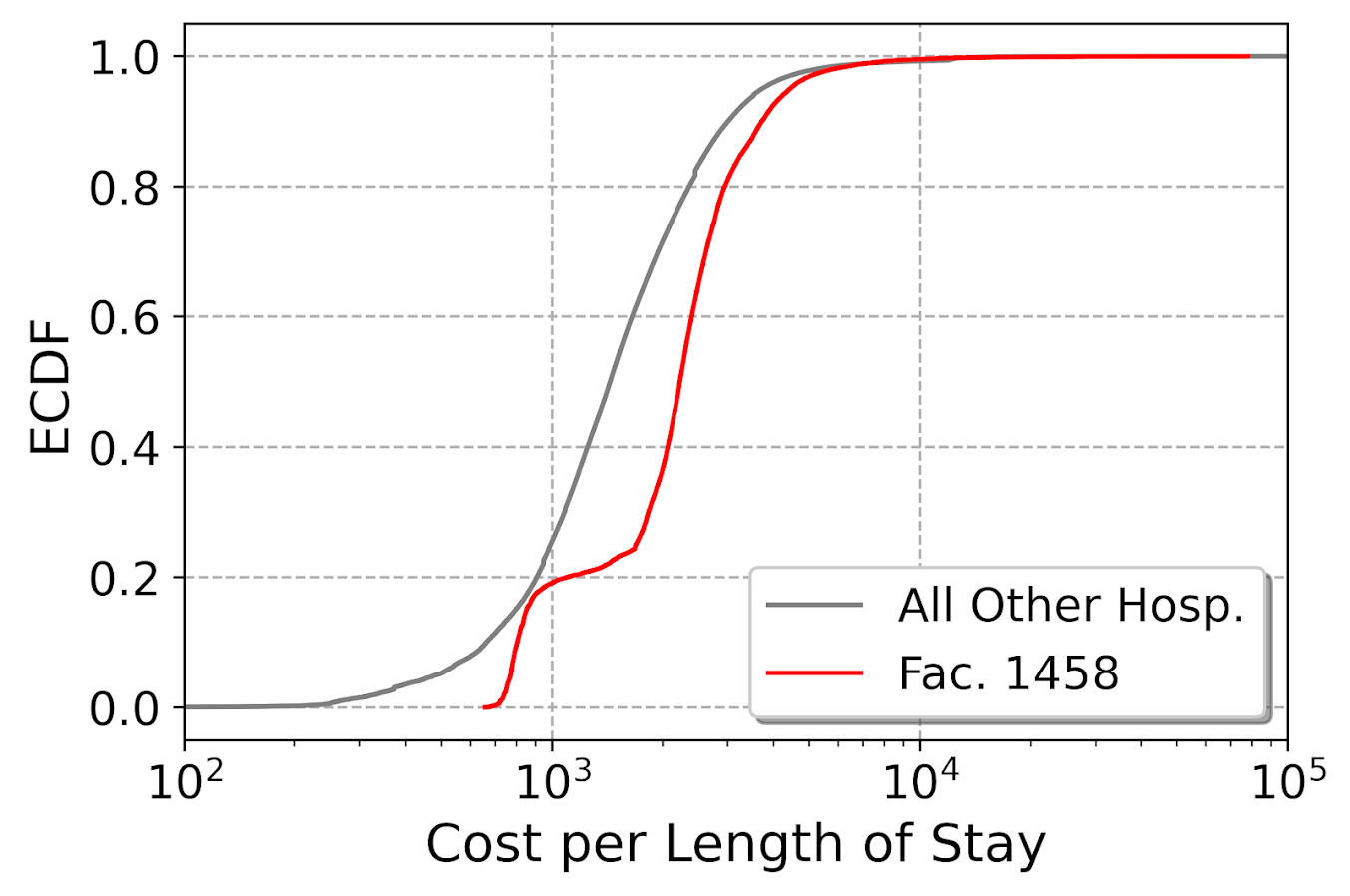For this example analysis, I am using data from New York State health insurance claims data (SPARC). I have posted python code to replicate here (note if you cannot access dropbox links, feel free to email and I will forward).

Here I am specifically analyzing medical, in-patient insurance claims (I dropped surgical claims) for around 300+ hospitals. There are quite a few claims in this data, over 2 million, and the majority of hospitals have plenty of claims to examine (so no hospitals with only 10 claims). I also specifically examine costs per length of stay. Initially I just examined costs, but will get to why I changed to the normalized version towards the end of the post.

# Analysis of CDF Outliers

So first what I did was attempt to do a leave-one-out type stat test using the Kolmogorov-Smirnov test. This is a test that looks at the maximum vertical difference between the CDFs I showed earlier. I should have known better though. Given this large of sample size, even with multiple comparison adjustments for false discovery rate, every hospital was considered an outlier. This is sort of the curse of null hypothesis significance testing, it is either underpowered, so you get null results when things should really be flagged, or with large samples everything is flagged.

So what I did first was make a graph of all the different CDFs for each individual hospital. You can see from this plot we have a mass of the distribution that looks very similar in shape, but is shifted left or right. (Hospitals can bill different values, i.e. casemix, so can have the same types of events but have different bills, so that is normal.) But then we have a few outliers really stick out.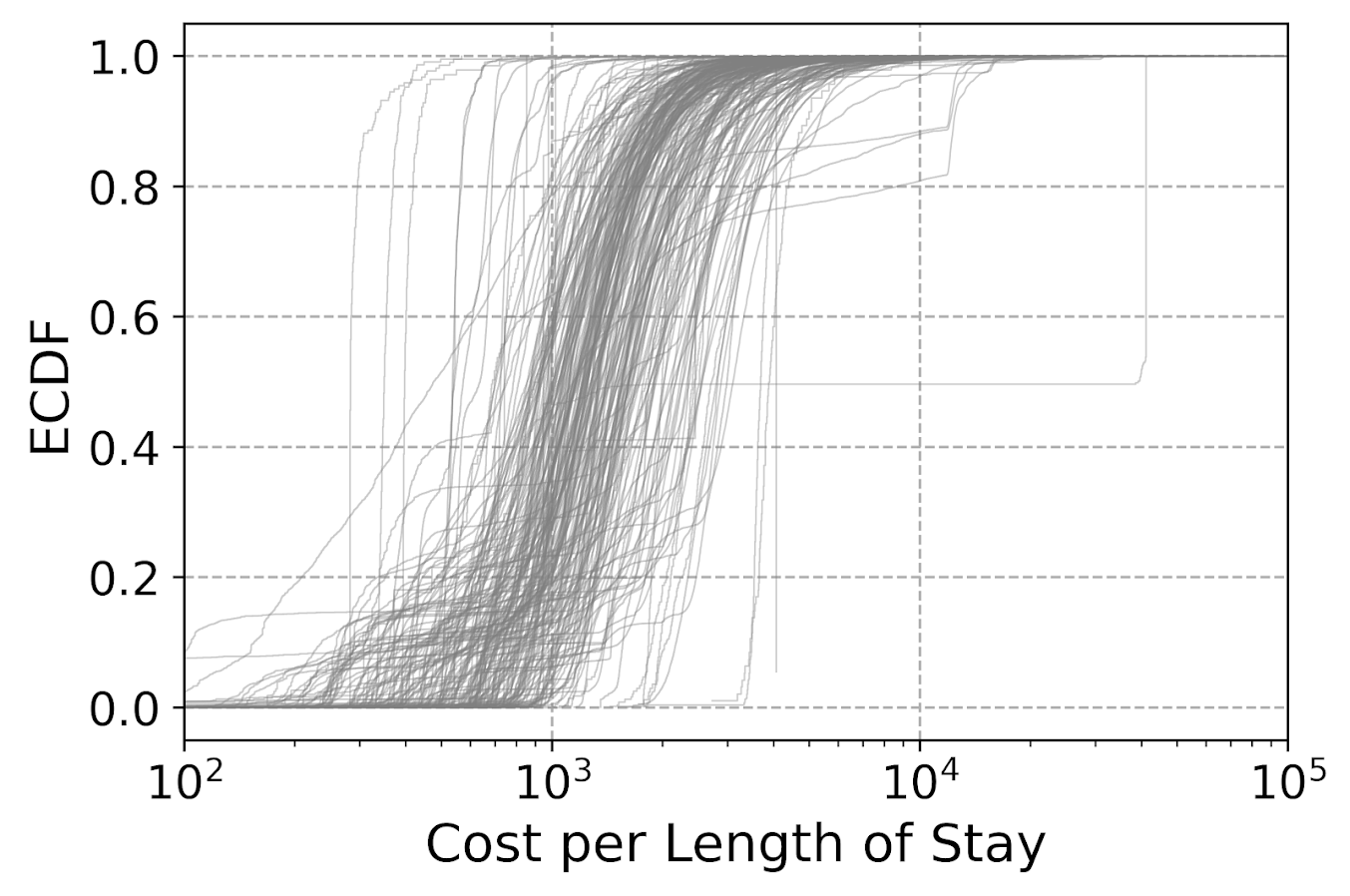To characterize the central mass in this image, what I did was calculate each empirical CDF for each hospital (over 300 in this sample). Then I estimated the CDF for each hospital at a sample of points logspace distributed between \$100 to \$100,000. Then I took the 90% distribution between the ECDF values. This is easier to show than to say, so in the below pic the grey area is the 90% region for the CDFs. Then you can do stats to see how hospitals may fall outside that band.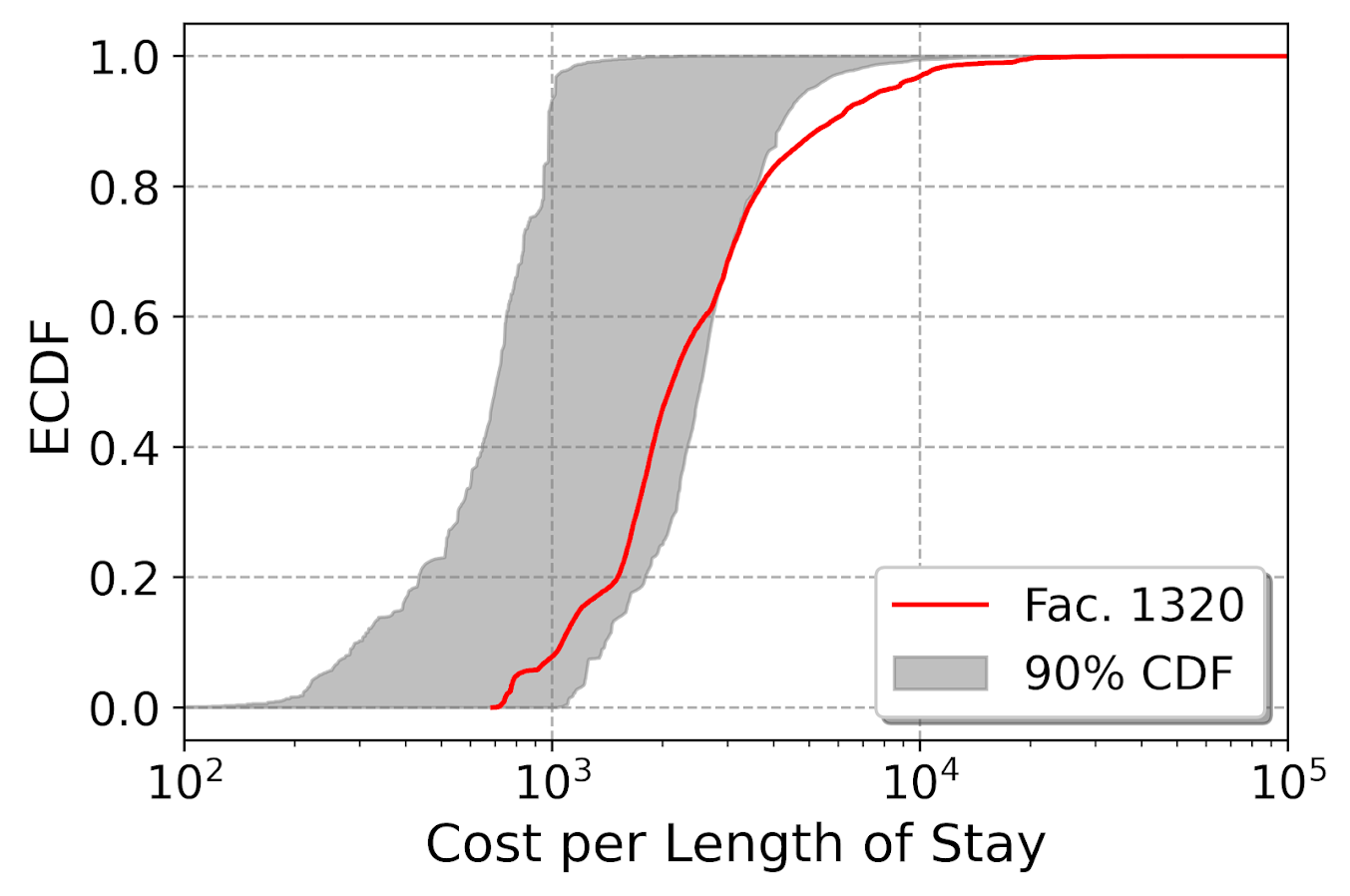So here 1320 is looking good until around 60% of the distribution, and then it is shifted right. There is a kink in the CDF as well, so this suggests really a set of different types of claims, and in that second group it is the outlier. 1320 was the hospital that had the most sample points outside of my grey coverage area, but you could also do outliers in terms of the distance between those two lines (again like a KS test stat), or in the area between those two lines (that is like a version of the Wasserstein distance only considering above/below moves). So here is the hospital that has the largest distance below the band (above the band signals that a hospital has lower claims on average):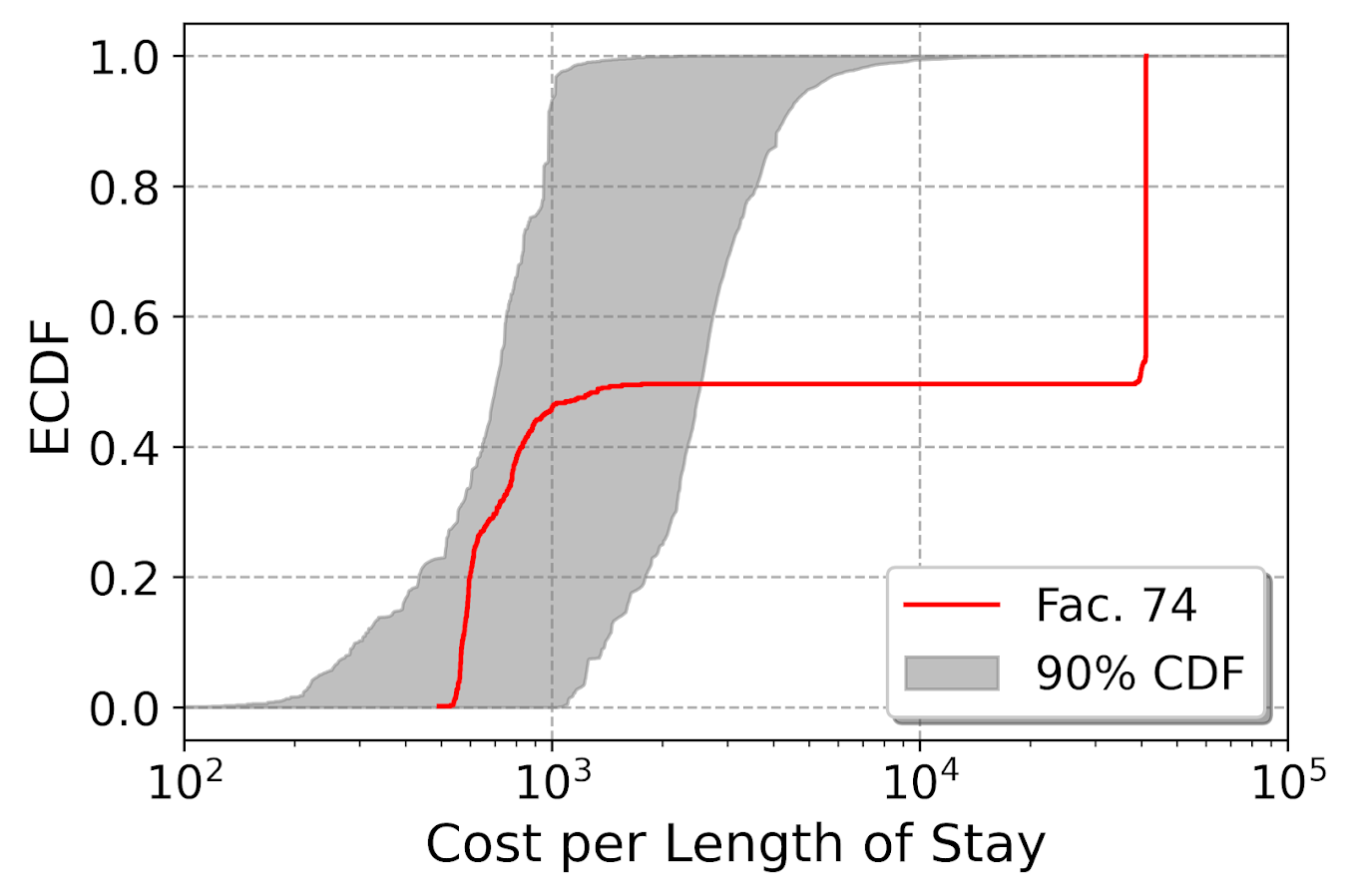Flat lines horizontally signal an absence of data, whereas vertical lines signal a set of claims with the exact same bill. So here we have a set of claims around \$1000 per day that look normal, then an abnormal absence of data from \$1,000 to \$10,000. Then a large spike of claims that end up being around \$45k per day.

So this is looking at the distribution relative to other hospitals, but a few examples I am familiar with look for these flat/vertical spikes in the CDF to identify fraud. Mike Maltz has an example of identifying collusion in bids. In another, Chris Stucchio identifies spikes in transaction data signaling potential fraud. Here I am just doing a test relative to other data to identify weird curves, not just flat lines though.

One limitation of this analysis I have conducted here is that it does not take into account the nature of the claims data. So say you had a hospital that specializes in cancer treatment, it may be totally normal for them to have claims that are higher value overall than a more typical hospital that spreads claims among a wider variety of types of visits/treatments. Initially I analyzed just the cost data, and it identified a few big outliers that ended up being hospice/nursing homes. So they had really high dollar value claims, but also really long stays. So when analyzing the claim per length of say, they were totally normal in that central mass.

So ultimately there could be other characteristics in the types of claims hospitals submit that could explain the weird CDF. One way to take that into account is to do a conditional model for the claims, and then do the ECDF tests on those conditional models. One way may be to look at the residuals for each individual hospital, another would be to draw a matched comparison sample. (Greg Ridgeway did this when examining police behavior in the NYPD.)

That would be like making a single comparison line (like my initial black/red line graph). So controlling the false discovery after that will be tough with larger samples (again the typical KS test, even with a matched sample, will likely always reject). So wondering if there is another machine learning way to identify outliers in CDF space, like a mashup of isolation forests and conditional density forests. Essentially I want to fit a model to draw those grey CDF bands, instead of relying on my sample of hospitals to draw the grey band in those latter plots.

# Regression with Simple Weights

I was reminded of this paper by Jung et al. on constructing simple rules via regression recently. So in the past few posts I have talked about how RTM (1,2) is aimed at making simple models. This is via variable selection and/or simplying the inputs to be binary yes/no. But in the end the final equation could be something like:

``log(Crime) = -0.56 + 0.6923*NearbyBars + 0.329*HighDensity311``

The paper linked above is about making the regression weights simple, so instead of a regression weight of 0.89728, you may just round the regression weight to 1. The Jung paper does a procedure where they use lasso regression and then round the weights. But there is a simpler approach IMO I will illustrate, just amend the lasso weights to push the coefficients to simple integers. (Also reminded by this example of using an iterative linear program to push weights to binary 0/1.)

So in lasso, you estimate your normal regression equation, but put a penalty on the weights that is typically something like `lambda*(sum(abs(reg_weights)) - 1)**2`. So if you have reg weights that add to more than 1, they are penalized by a particular amount (the lambda is a tuner to make the penalty higher/lower). And in the iterative algorithm to minimize your loss function plus this added penalty, it will converge to regression weights that meet the criteria of in total summing to around 1. Not exactly 1 but close.

You can however swap out that penalty term with whatever you want (or add to it additional penalties). I will show an example of using a penalty term to push regression coefficients towards integer values, creating simple regression weights.

# Why Simple Models?

Dan Simpson has a good blog post of the Jung paper and why simple models are sometimes preferable (and I also have a comment why simple models like this tend to work out well for CJ datasets). But here are few quick examples why you might want a simple model results.

Example 1: If you have people in the field who are tabulating data and making quick decisions, it may be they need to use pen/paper and make a quick decision. No time to input results into a computer and pop out a prediction. Imagine a nurse in the ER, or even your general practitioner. There may be quite a bit of utility in making a simple check list that says `if +4 on this scale, do a more intensive treatment`.

Example 2: You have a complicated, large database. It is easier to create a simple predictive model in SQL to serve up predictions (either because of latency or because of the complexity of the data pipeline). Instead of a complicated random forest, a linear regression with simple weights will be much easier to implement.

Example 3: Transparency. Complicated models are more difficult to understand and monitor. If you have a vested interest in presenting the model to outside parties, it may make sense to sacrifice some accuracy to make the model more interpretable. Also similar to lasso, I suspect these simple weights will reduce the variance of predictions.

The reason that these simple weights work well in practice for many social science examples you could interpret either in a good light or a bad one. For the half-empty interpretation, our models are not well identified – we can literally swap out various weights in our regression equation and get near similar predictions. So it is fools errand to try to find the regression equation that describes the underlying system. But you can flip that around as well, we don’t even need to find the perfect equation, we can identify quite a few good predictive equations. And why not pick a good equation that is easier to interpret?

# Pytorch Example

The example set of code here is very simple, so I will just put the python code entirely in this post. First I import my libraries I am using and change my directory.

``````############################################
import os
import torch
import statsmodels.api as sm
import statsmodels.formula.api as smf
import pandas as pd
import numpy as np

my_dir = r'D:\Dropbox\Dropbox\Documents\BLOG\regression_simpleweights\analysis'
os.chdir(my_dir)
############################################``````

Next I read in the data, which I have previously used as an example in prior blog posts on doctor visits for medicare patients. One thing to note here, is that I rescale the independent variables I am using to min/max. So the age variable instead of going from 65-90 like in the original data, now is scaled to be between 0/1. This is a problem intrinsic to lasso as well, in that you can change the scale of the input variables and it changes the weights. Here with the original data, the education variable has a tiny regression coefficient (0.2), but is highly stat significant. So without rescaling that variable, the model said to hell with your penalty and still converged to a solution of that regression weight is 0.2. If you divide the education variable by 5 though, the corresponding regression weight would change to around 1.

``````###########################################
#Data from Stata, https://www.stata-press.com/data/r16/gsem_mixture.dta
#see pg 501 https://www.stata.com/manuals/sem.pdf

visit_dat = pd.read_stata('gsem_mixture.dta')
y_dat = visit_dat[['drvisits']]
x_vars = ['private','medicaid','age','educ','actlim','chronic']
#rescaling variables to 0/1
x_dat = visit_dat[x_vars]
visit_dat[x_vars] = (x_dat - x_dat.min(axis=0)) / ( x_dat.max(axis=0)  - x_dat.min(axis=0) )
x_dat = visit_dat[x_vars] #intentional not a copy
###########################################``````

Now in the next part, I estimate the default linear regression model using statsmodels for reference. Then I stuff the results into pytorch tensors (which I will use later as default starting points for the pytorch estimates). Below is a pic of the resulting summary for the regression model (with the scaled variables, so is slightly different than my prior post).

``````###########################################
#Estimating the same model in statsmodel
#for confirmation of the result

stats_mod = smf.ols(formula='drvisits ~ private + medicaid + age + educ + actlim + chronic',
data=visit_dat)
sm_results = stats_mod.fit()
print(sm_results.summary())

#What is the mean squared error
pred = sm_results.get_prediction().summary_frame()
print( ((y_dat['drvisits'] - pred['mean'])**2).mean() )
#169513.0122252265 for sum
#46.10 for mean

#for setting default initial weights
coef_table = sm_results.params
int_ten = torch.tensor([coef_table], dtype=torch.float, requires_grad=True)
coef_ten = torch.tensor(pd.DataFrame(coef_table[1:]).T.to_numpy(), dtype=torch.float, requires_grad=True)
###########################################``````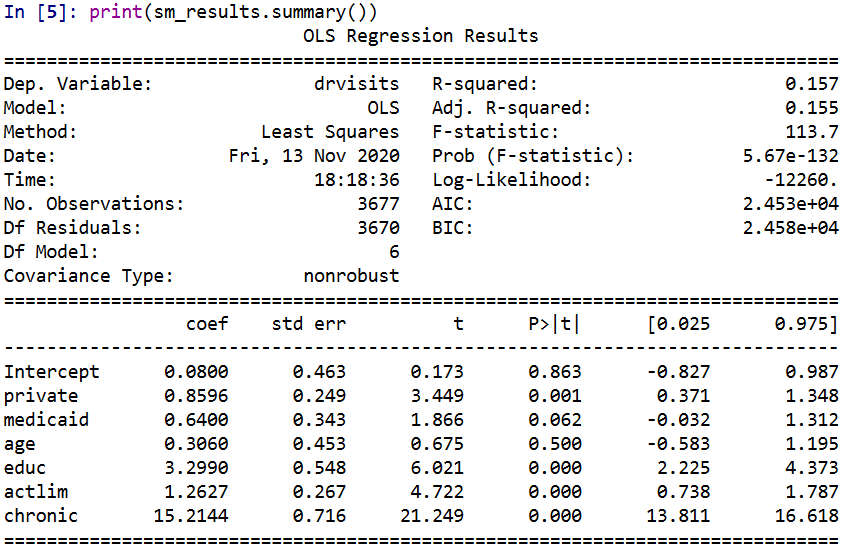Now creating the pytorch model is quite simple. For linear regression it is just one linear layer, and then setting the loss function to mean squared error. Then I create my own simple weight penalization factor in the `simp_loss` function. This takes the regression weights (not including the bias/intercept term), takes the difference between the observed weight and the rounded weight, takes the absolute value and sums those absolute values up. Then in the loop when I am fitting the model, you can see the `loss = criterion(y_pred, y_ten) + 0.4*simp_loss(model)` line. For the usual linear regression, it would just be the first criterion term. Here to add in the penalty term is super simple in pytorch, you just add it to the loss. (And you can incorporate additional penalities, the same way ala elastic-net. The Jung paper they put a penalty on the sum of coefficients as per the original lasso as well.)

Then the final part of the code after the loop is just putting the coefficients in a nicer data frame to print. And below the code snippet are the results.

``````###########################################
#Now estimating OLS model with simple coefficient
#Penalities in Pytorch

torch.manual_seed(10)

model = torch.nn.Sequential(
torch.nn.Linear(len(x_vars),1,bias=True)
)

##Initializing weights
#with torch.no_grad():
#    model.weight = torch.nn.Parameter(coef_ten)
#    model.bias = torch.nn.Parameter(int_ten)

x_ten = torch.tensor( x_dat.to_numpy(), dtype=torch.float)
y_ten = torch.tensor( y_dat.to_numpy(), dtype=torch.float)

criterion = torch.nn.MSELoss(reduction='mean')
optimizer = torch.optim.Adam(model.parameters(), lr=1e-4)

def simp_loss(mod):
dif = mod.weight - torch.round(mod.weight)
return dif.abs().sum()

for t in range(100000):
#Forward pass
y_pred = model(x_ten)
#Loss
loss = criterion(y_pred, y_ten) + 0.4*simp_loss(model)
if t % 1000 == 99:
print(f'iter: {t}, loss: {loss.item()}')
#Zero gradients
optimizer.zero_grad()
loss.backward()
optimizer.step()

#Making a nice dataframe of coefficients

coef_vars = ['Inter'] + x_vars
vals = list(model.bias.detach().numpy()) + list(model.weight.detach().numpy()[0,:])
res = pd.DataFrame(zip(coef_vars, vals), columns=['Var','Coef'])
print( res )
###########################################``````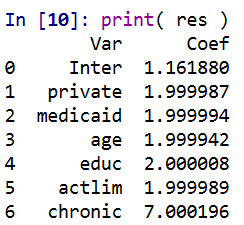Here I did not round the coefficients, so you can see that they are not exactly integer values, but are very close. So this will result in a lower loss than taking the usual linear regression coefficients and rounding them like in the noted Jung paper. It is a more direct approach. Also note that the intercept is not close to an integer value. I did not include the intercept in my penalty term. You could if you wanted to, but for most examples I don’t think it makes much sense to do that.

But one of the things that I have noticed playing around with pytorch more is that it is very difficult to get random initialized weights to converge to the same solution. That identification problem I mentioned earlier. One way is instead of using random initialized weights, is to initialize them to some reasonable values. If you uncomment the lines `with torch.no_grad():` in the above code and initialize the weights to start from the unregularized OLS solution, it converges much faster, has a slightly smaller mean square error term, and results in these effects: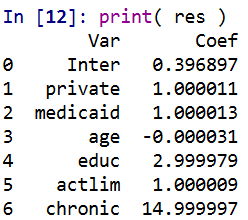So you can see in that solution it is exactly the same as rounding the initial OLS solution (ignoring the intercept again). But that may not always be the case. For example if actlim (activity limitations) and educ (education) had a very high correlation, it may be rounding both down is too big a hit to the fit of the equation, so one may go down and one go up. (You need to estimate the equation to know if things like that will occur.)

And that is all folks! While if I were sharing this more broadly, I would likely make a statsmodel like interface (and it appears they use cvxopt under the hood) instead of pytorch, it is very simple to amend pytorch to return simple weights, just add in the penalty to the loss function. Works the same way for lasso/ridge as it does for the simple weights example I give here.

Next up I want to try to figure out autograd in pytorch good enough to give standard errors for these various regression models I am estimating. While I don’t think hypothesis testing makes sense for these various models I am sharing, seeing a standard error that is very high may have prognostic value. In this case, if you had a very high standard error relative to the simple coefficient, it might suggest you should rescale the variable a different way or drop it entirely.

Also for this example, to be simple in the field it would not only need simple coefficients, but simple inputs as well. Wondering if there is a way to add in threshold layers in deep learning to automatically figure out the best way to make the inputs binary (e.g. above 70, educ below 10, etc.) instead of doing min/max scaling of the inputs.

• ## Follow Blog via Email

Enter your email address to follow this blog and receive notifications of new posts by email.

Join 257 other followers